Four-Space Visualization of 4D Objects by
Steven Richard Hollasch

A Thesis Presented in Partial Fulfillment
of the Requirements for the Degree
Master of Science

## Arizona State UniversityAugust 1991

Supervisory Committee:

• Dr. Thomas Foley
• Dr. Gregory Nielson
• Dr. Gerald Farin

# Abstract

In the field of scientific visualization, the term “four dimensional visualization” usually refers to the process of rendering a three dimensional field of scalar values. While this paradigm applies to many different data sets, there are also uses for visualizing data that correspond to actual four-dimensional structures. Four dimensional structures have typically been visualized via wireframe methods, but this process alone is usually insufficient for an intuitive understanding; all but the most simple datasets easily overwhelm the viewer. This research covers the visualization of four dimensional objects through wireframe methods with extended visualization cues, and through raytracing methods. Both the wireframe and raytracing methods employ true four-space viewing parameters and geometry. The raytracing approach easily solves the hidden surface and shadowing problems of 4D objects, and yields an image in the form of a three-dimensional field of RGB values, which can be rendered with a variety of existing methods. The 4D raytracer also supports true four-dimensional lighting, reflections and refractions.

# Acknowledgements

In appreciation of the time, effort and patience extended to me in the pursuit of this thesis, I would like to thank my committee chairman, Dr. Thomas Foley. In addition to his guidance in the topic of this research, he has also been unfailing in helping me get the resources and information I needed to complete both this research and this paper. I would also like to acknowledge and thank Dr. Gregory Nielson and Dr. Gerald Farin for being on my thesis committee and offering their observations and suggestions.

Thanks are also due to Kevin Price for letting me use his Amiga and helping me produce animations of the raytrace data that aided my understanding of the process and nature of four-dimensional raytracing.

Finally, I would like to thank my family for their patience, confidence, enthusiasm and financial support.

# List of Figures

 3.1 The 3D Viewing Vectors and From, To Points 3.2 The Resulting View From Figure 3.1 3.3 The 3D Viewing Vectors and Viewing Frustum 3.4 The 4D Viewing Vectors and Viewing Frustum 4.1 3D Eye Coordinates 4.2 3D Perspective Projection in Eye Coordinates 4.3 4D Eye Coordinates 4.4 4D Perspective Projection in Eye Coordinates 4.5 The 4-Cube with Various 4D and 3D Projections 4.6 Another View of The 4-Cube with Various 4D and 3D Projections 4.7 4D Rotation of the 4-Cube 4.8 The 4-Cube With All Edges Rendered in One Color 4.9 The Dual of The 4-Cube With All Edges Rendered in One Color 4.1 The 4-Cube Rendered With Multiple Edge Colors 4.11 The Dual of The 4-Cube Rendered With Multiple Edge Colors 4.12 The 4-Cube Rendered With Depth-Cueing 4.13 The Dual of The 4-Cube Rendered With Depth-Cueing 4.14 A 4D Curve on a 4-Sphere 4.15 A 4D Curve on a 4-Sphere with Poor Parameterization 5.1 A 2x2x2 4D Raytrace Grid 5.2 Ray-Object Reflection 5.3 Components of Illumination 5.4 Sliced 4D Image Cube of Random 4-Sphere Distribution 5.5 Sliced Image of 16 4-Spheres Placed at 4-Cube Vertices 5.6 Sliced Image of 16 4-Tetrahedrons Placed at 4-Cube Vertices

# Chapter 1Introduction

The display of four-dimensional data is usually accomplished by assigning three dimensions to location in three-space, and the remaining dimension to some scalar property at each three-dimensional location. This assignment is quite apt for a variety of four-dimensional data, such as tissue density in a region of a human body, pressure values in a volume of air, or temperature distribution throughout a mechanical object.

While there exist a number of methods to approach the visualization of three-dimensional scalar fields ([Chen 85], [Drebin 88], [Kajiya 84], [Lorensen 87], and [Sabella 88] are good examples), there are few methods that are effective on true four-dimensional data, where the data do not represent a three-dimensional scalar field.

This paper approaches the problem of displaying 4D objects as physical models through two main approaches: wireframe methods and raytracing. Both of these methods employ true four-dimensional viewpoints and viewing parameters, and light (or depthcue) the rendered objects from four-space.

## 1.1 Background

The tremendous difficulty of visualizing true four-space data lies in the fact that there are no solid paradigms for three-space creatures such as ourselves. This difficulty is best understood in imagining the plights of two-space creatures who try to comprehend our three-space world (see [Abbott 52] or [Dewdney 84] for explorations of this idea).

The method we use to comprehend three-dimensional scenes is quite complex, since each eye is presented with only the two-dimensional projection of the three-dimensional scene. There are several methods we employ to convert these 2D projections to an imaginary 3D model. These methods include focusing (of limited help but useful when viewing with a single eye), parallax (deriving depth through binocular vision), application of object knowledge to understand different views, and motion to derive depth information.

These methods of visualizing 3D objects are very strong, and are usually quite accurate. When dealing with a single rendered 2D projection, however, the image becomes a bit more difficult to comprehend, because the viewer can no longer use focusing or parallax to extract information from the scene.

Usually, however, rendered 2D projections are quite intelligible because these projections involve objects or structures familiar to the viewer, and because shadows and highlights also help the viewer to extract depth information. The main thing that aids our understanding of a scene when given only 2D projections is our experience and intuitive understanding of the 3D world. This additional understanding helps us to intuitively reconstruct the original 3D scene, and to accurately guess the portions for which we have no visual information.

If we are deprived of this intuition and experience, then reconstructing the original scene from a projection is very difficult. This is what makes visualizing 4D data such a complex task. In fact, when rendering a 4D scene, not one but two dimensions of the original data are lost; one can think of a screen image of 4D data as a projection of the projection of the scene. This further loss of information, coupled with our lack of intuitive understanding, demands that the 4D visualization methods provide additional information, usually in the form of motion, multiple views or other visual cues. These techniques will be presented in more detail later in this paper.

## 1.2 Previous Work

The idea of understanding four-dimensional space is at least as old as the nineteenth century, and has been studied mathematically at least as early as the 1920's. Understanding four-space has also been explored in texts that attempt to model two-dimensional creatures and their perceptions of three-space (see [Abbott 52] and [Dewdney 84]). Studying the difficulties of two-space creatures who attempt to understand three-space often yields insight into the problem of understanding four-space from our three-dimensional world.

The task of viewing four-space structure has been explored as early as [Noll 67], who rendered four-dimensional wireframes with 4D perspective projection. Noll was limited in his exploration of four-dimensional structures by the technology of that time; his method consisted of generating pictures via plotter and then transferring each drawing onto film. The movies he produced yielded a great amount of insight into the structure of various four-dimensional objects. However, the lack of interaction was certainly a significant hindrance.

In 1978, David Brisson ([Brisson 78]) presented hyperstereograms of 4D wireframes. These hyperstereograms are unconventional in the sense that the viewer must rotate the hyperstereograms in order to resolve the second degree of parallax of the 4D view. These hyperstereograms are very difficult to view, but do provide another method of understanding the four-dimensional structures.

In the early 1980's, Thomas Banchoff (who is heavily involved in the visualization of four-space) rendered hypersphere “peels” ([Dewdney 86] and [Banchoff 90]) which resulted in some beautiful images of their rotation in four-space.

Several people have rendered four-dimensional objects by producing the three-dimensional slices of the object; this is presented in [Banchoff 90].

Rendering solid four-dimension objects yields a three-dimensional “image”, rather than the two-dimensional image of a three-dimensional object. [Steiner 87] and [Carey 87] use scanplane conversion to render four-dimensional objects into three-dimensional voxel fields. Both approaches also tackle the difficult problem of hidden volume removal in four-dimensional views. The resulting fields are then displayed with semi-transparent voxels in order to view the internal structure of the three-dimensional projections.

## 1.3 Overview of This Research

This research builds on the existing wireframe display techniques and tackles the visualization of solid four-dimensional objects via raytracing techniques.

The wireframe viewer takes as input a list of four-space vertices and a list of edges between pairs of these vertices. It produces a single image which is the 2D projection of the 4D scene, and which may be interactively rotated, depthcued, and parallel- or perspective-projected.

The limitations of the 4D wireframe viewer are pretty much the same as those for a 3D wireframe viewer: the lack of surface information, the multiple ambiguities of a wireframe view, and the fact that all objects must be decomposed to vertices and edges.

The significant advantage of the wireframe viewer is that image display is quite fast, especially when compared to raytracing methods. This speed of display allows for interactive rotation of the 4D object, which greatly aids in an understanding of the object and its relationship to the rest of the scene. Another advantage is that the wireframe viewer is able to display curves in four-space.

The 4D raytracer solves the hidden volume and lighting problem in a very straight-forward manner (as for 3D). It takes an input file of 4D object information and probes the scene that contains these objects. The implemented 4D raytracer handles three primitives: hyperspheres, tetrahedra, and parallelepipeds, although the methods presented in this paper apply to a much broader class of objects.

The output of the 4D raytracer is a gridded 3D volume of RGB triples, analogous to the gridded 2D volume of RGB data produced by a 3D raytracer. The disadvantages of the raytracer include the increased rendering time and the resultant 3D volume, which must be further rendered with other methods.

The main advantages of the raytracer include the fact that the rendered image has hidden volumes, shadows, highlights, reflections and other artifacts that aid the understanding of the scene.

## 1.4 Contents of This Paper

Chapter 2 covers the four-dimensional geometry that is used in this research, and focuses on vector operations and 4D rotations. The 3D and 4D viewing models are presented on a functional level in Chapter 3 and implemented in detail in Chapters 4 and 5. Chapter 4 covers the rendering of 4D data via wireframe methods. It describes the method of projecting the image from four-space to three-space, and then from three-space to the 2D viewport. It also covers the implementation of 4D object rotation and other wireframe visual aids. The four-dimensional raytracing method is presented in Chapter 5; this includes the generation of the ray target grid, the intersection algorithms used for the fundamental 4D objects, and the methods of visualizing the resulting 3D “image” of RGB data. Finally, Chapter 6 provides a conclusion to this research. It includes notes on the research in general, suggestions for further research and exploration, and provides a few comments on the implementation of the programs discussed in this paper.

# Chapter 2Four Dimensional Geometry

Many of the underlying mathematical operations used in the 3D rendering process extend effortlessly to four dimensions. The rotation and cross-product operations, however, do not extend easily or intuitively; these are presented here before continuing with the rest of this paper.

## 2.1 Vector Operations and Points in Four-Dimensional Space

For the most part, vector operations in four space are simple extensions of their three-space counterparts. For example, computing the addition of two four-vectors is a matter of forming a resultant vector whose components are the sum of the pairwise coordinates of the two operand vectors. In the same fashion, subtraction, scaling, and dot-products are all simple extensions of their more common three-vector counterparts.

In addition, operations between four-space points and vectors are also simple extensions of the more common three-space points and vectors. For example, computing the four-vector difference of four-space points is a simple matter of subtracting pairwise coordinates of the two points to yield the four coordinates of the resulting four-vector.

For completeness, the equations of the more common four-space vector operations follow. In these equations, U = <U₀, U₁, U₂, U₃> and V = <V₀, V₁, V₂, V₃> are two source four-vectors and k is a scalar value:

U + V = <U₀ + V₀, U₁ + V₁, U₂ + V₂, U₃ + V₃> U − V = <U₀ − V₀, U₁ − V₁, U₂ − V₂, U₃ − V₃> kV = <kU₀, kU₁, kU₂, kU₃> U⋅V = U₀V₀ + U₁V₁ + U₂V₂ + U₃V₃

The main vector operation that does not extend trivially to four-space is the cross product. A three-dimensional space is spanned by three basis vectors, so the cross-product in three-space computes an orthogonal three-vector from two linearly independent three-vectors. Hence, the three-space cross product is a binary operation.

In N-space, the resulting vector must be orthogonal to the remaining N−1 basis vectors. Since a four-dimensional space requires four basis vectors, the four-space cross product requires three linearly independent four-vectors to determine the remaining orthogonal vector. Hence, the four-space cross product is a trinary operation; it requires three operand vectors and yields a single resultant vector. In the remainder of this paper, the four-dimensional cross product will be represented in the form X₄(U,V,W).

To find the equation of the four-dimensional cross product, we must first establish criteria of the cross product. These are as follows:

1. If the operand vectors are not linearly independent, the cross product must be the zero vector.
2. If the operand vectors are linearly independent, then the resultant vector must be orthogonal to each of the operand vectors.
3. The four-space cross product preserves scaling, i.e. for any scalar k: kX₄(U,V,W) = X₄(kU,V,W) = X₄(U,kV,W) = X₄(U,V,kW)
4. Changing the order of two of the operands results only in a sign change of the resultant vector.

It turns out that a somewhat simple-minded approach to computing the four-dimensional cross product is the correct one. To motivate this idea, we first consider the three-dimensional cross product. The 3D cross product can be thought of as the determinant of a 3×3 matrix whose entries are as follows:

⎡ i j k ⎤ X₃(U,V) = ⎢ U₀ U₁ U₂ ⎥ ⎢ V₀ V₁ V₂ ⎥ ⎣ ⎦

where U and V are the operand vectors, and i, j & k represent the unit components of the resultant vector. The determinant of this matrix is

i (U₁V₂ − U₂V₁) − j (U₀V₂ − U₂V₀) + k (U₀V₁ − U₁V₀)

which is the three-dimensional cross product. Using this idea, we'll form the analogous 4×4 matrix, and see if it meets the four cross product properties listed above:

⎡ i j k l ⎤ X₄(U,V,W) = ⎢ U₀ U₁ U₂ U₃ ⎥ ⎢ V₀ V₁ V₂ V₃ ⎥ ⎢ W₀ W₁ W₂ W₃ ⎥ ⎣ ⎦ [2.1a]

The determinant of this matrix is

⎢ U₁ U₂ U₃ ⎥ ⎢ U₀ U₂ U₃ ⎥ ⎢ U₀ U₁ U₃ ⎥ ⎢ U₀ U₁ U₂ ⎥ i ⎢ V₁ V₂ V₃ ⎥ − j ⎢ V₀ V₂ V₃ ⎥ + k ⎢ V₀ V₁ V₃ ⎥ − l ⎢ V₀ V₁ V₂ ⎥ ⎢ W₁ W₂ W₃ ⎥ ⎢ W₀ W₂ W₃ ⎥ ⎢ W₀ W₁ W₃ ⎥ ⎢ W₀ W₁ W₂ ⎥ ⎢ ⎥ ⎢ ⎥ ⎢ ⎥ ⎢ ⎥ [2.1b]

If the operand vectors are linearly dependent, then the vector rows of the 4×4 matrix will be linearly dependent, and the determinant of this matrix will be zero. This satisfies the first condition. The third condition is also satisfied, since a scalar multiple of one of the vectors yields a scalar multiple of one of the rows of the 4×4 matrix. This results in a determinant that is scaled by that factor, so condition three is also met.

The fourth condition falls out as a property of determinants, i.e. when two rows of a determinant matrix are interchanged, only the sign of the determinant changes. Hence, the fourth condition is also met.

The second condition is proven by calculating the dot product of the resultant vector with each of the operand vectors. These dot products will be zero if and only if the resultant vector is orthogonal to each of the operand vectors.

The dot product of the resultant vector X4(U,V,W) with the operand vector U is the following (refer to equation [2.1b]):

U·X₄(U,V,W) = ⎢U₁ U₂ U₃⎥ ⎢U₀ U₂ U₃⎥ ⎢U₀ U₁ U₃⎥ ⎢U₀ U₁ U₂⎥ U₀⎢V₁ V₂ V₃⎥ − U₁⎢V₀ V₂ V₃⎥ + U₂⎢V₀ V₁ V₃⎥ − U₃⎢V₀ V₁ V₂⎥ ⎢W₁ W₂ W₃⎥ ⎢W₀ W₂ W₃⎥ ⎢W₀ W₁ W₃⎥ ⎢W₀ W₁ W₂⎥ ⎢ ⎥ ⎢ ⎥ ⎢ ⎥ ⎢ ⎥

This dot product can be rewritten as the determinant

⎢ U₀ U₁ U₂ U₃ ⎥ ⎢ U₀ U₁ U₂ U₃ ⎥ ⎢ V₀ V₁ V₂ V₃ ⎥ ⎢ W₀ W₁ W₂ W₃ ⎥ ⎢ ⎥,

which is zero, since the first two rows are identical. Hence, the resultant vector X₄(U,V,W) is orthogonal to the operand vector U. In the same way, the dot products of V·X₄(U,V,W) and W·X₄(U,V,W) are given by the determinants

⎢ V₀ V₁ V₂ V₃ ⎥ ⎢ W₀ W₁ W₂ W₃ ⎥ ⎢ U₀ U₁ U₂ U₃ ⎥ ⎢ U₀ U₁ U₂ U₃ ⎥ ⎢ V₀ V₁ V₂ V₃ ⎥ and ⎢ V₀ V₁ V₂ V₃ ⎥ ⎢ W₀ W₁ W₂ W₃ ⎥ ⎢ W₀ W₁ W₂ W₃ ⎥ ⎢ ⎥ ⎢ ⎥,

which are each zero.

Therefore, the second condition is also met, and equation [2.1a] meets all four of the criteria for the four-dimensional cross product.

Since the calculation of the four-dimensional cross product involves 2x2 determinants that are used more than once, it is best to store these values rather than re-calculate them. The following algorithm uses this idea.

// Cross4 computes the four-dimensional cross product of the three vectors // U, V and W, in that order. It returns the resulting four-vector. Vector4 *Cross4 (Vector4 *result, Vector4 U, Vector4 V, Vector4 W) { double A, B, C, D, E, F; // Intermediate Values // Calculate intermediate values. A = (V * W) - (V * W); B = (V * W) - (V * W); C = (V * W) - (V * W); D = (V * W) - (V * W); E = (V * W) - (V * W); F = (V * W) - (V * W); // Calculate the result-vector components. *result = (U * F) - (U * E) + (U * D); *result = - (U * F) + (U * C) - (U * B); *result = (U * E) - (U * C) + (U * A); *result = - (U * D) + (U * B) - (U * A); return result; }

## 2.2 Rotations in Four Dimensions

Rotation in four space is initially difficult to conceive because the first impulse is to try to rotate about an axis in four space. Rotation about an axis is an idea fostered by our experience in three space, but it is only coincidence that any rotation in three-space can be determined by an axis in three-space.

For example, consider the idea of rotation in two space. The axis that we rotate “about” is perpendicular to this space; it isn't even contained in the two space. In addition, given an origin of rotation and a destination point in three space, the set of all rotated points for a given rotation matrix lie in a single plane, just like the case for two space.

Rotations in three-space are more properly thought of not as rotations about an axis, but as rotations parallel to a 2D plane. This way of thinking about rotations is consistent with both two space (where there is only one such plane) and three space (where each rotation “axis” defines the rotation plane by coinciding with the normal vector to that plane).

Once this idea is established, it is easy to construct the basis 4D rotation matrices, since only two coordinates will change for a given rotation. There are six 4D basis rotation matrices, corresponding to the XY, YZ, ZX, XW, YW and ZW planes. These are given by (using angle T):

⎡ cosT sinT 0 0 ⎤ ⎡ 1 0 0 0 ⎤ ⎡ cosT 0 −sinT 0 ⎤ ⎢−sinT cosT 0 0 ⎥ ⎢ 0 cosT sinT 0 ⎥ ⎢ 0 1 0 0 ⎥ ⎢ 0 0 1 0 ⎥ ⎢ 0 −sinT cosT 0 ⎥ ⎢ sinT 0 cosT 0 ⎥ ⎢ 0 0 0 1 ⎥ ⎢ 0 0 0 1 ⎥ ⎢ 0 0 0 1 ⎥ ⎣ ⎦ ⎣ ⎦ ⎣ ⎦ XY Plane YZ Plane ZX Plane ⎡ cosT 0 0 sinT ⎤ ⎡ 1 0 0 0 ⎤ ⎡ 1 0 0 0 ⎤ ⎢ 0 1 0 0 ⎥ ⎢ 0 cosT 0 −sinT ⎥ ⎢ 0 1 0 0 ⎥ ⎢ 0 0 1 0 ⎥ ⎢ 0 0 1 0 ⎥ ⎢ 0 0 cosT −sinT ⎥ ⎢−sinT 0 0 cosT ⎥ ⎢ 0 sinT 0 cosT ⎥ ⎢ 0 0 sinT cosT ⎥ ⎣ ⎦ ⎣ ⎦ ⎣ ⎦ XW Plane YW Plane ZW Plane

# Chapter 3 Overview of Visualization in Three and Four Space

Before describing the rendering methods for four-space visualization, we need to establish a viewing model that adequately describes a view of and in four space. This view needs to account for position of the viewpoint, direction of view, and the orientation of the scene from the viewpoint (or, conversely, the orientation of the viewer).

This chapter contains only the concepts of viewing in three- and four-space; the mathematical and implementation details are presented in chapter 4 and chapter 5.

## 3.1 Viewing in Three-Space

Before attacking the four dimensional viewing model, let's review the viewing model for three dimensions (presented in [Foley 87]).

The first thing to establish is the viewpoint, or viewer location. This is easily done by specifying a 3D point in space that marks the location of the viewpoint. This is called the from-point or viewpoint.

The next thing to establish is the line of sight (where we're looking). This can be done by either specifying a line-of-sight vector, or by specifying a point of interest in the scene. The point-of-interest method has several advantages. One advantage is that the person doing the rendering usually has something in mind to look at, rather than some particular direction. It also has the advantage that you can “tie” this point to a moving object, so we can easily track the object as it moves through space. This point of interest is called the to-point.

Now that we've established the line of sight, we need to pin down the orientation of the viewer/scene. This parameter will keep us from looking at a scene upside down, for example. To do this, we specify a vector that will point straight up after being projected to the viewing plane. This vector is called the up-vector. Since the up-vector specifies the orientation of the viewer about the line-of-sight, the up-vector must not be parallel to the line of sight. The viewing program uses the up-vector to generate a vector orthogonal to the line of sight and that lies in the plane of the line of sight and the original up-vector. See figure 3.1 for a diagram of the 3D viewing vectors.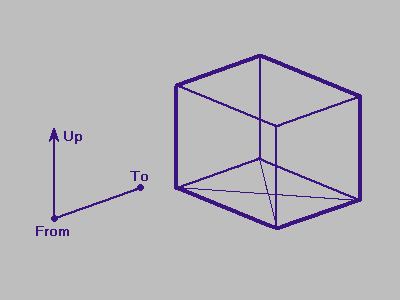Figure 3.1
The 3D Viewing Vectors and From, To Points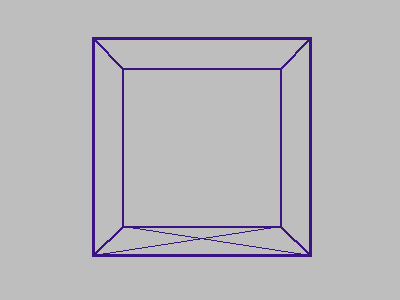Figure 3.2
The Resulting View From Figure 3.1

If we're going to use perspective projection, we need to specify the amount of perspective, or “zoom”, that the resultant image will have. This is done by specifying the angle of the viewing cone, also known as the viewing frustum. The viewing frustum is a rectangular cone in three-space that has the from-point as its tip, and that encloses the projection rectangle, which is perpendicular to the cone axis. The angle between opposite sides of the viewing frustum is called the viewing angle. It is generally easier to let the viewing angle specify the angle for one dimension of the projection rectangle, and then to tailor the angle of the perpendicular angle of the viewing frustum to match the other dimension of the projection rectangle.

The greater the viewing angle, the greater the amount of perspective (wide-angle effect), and the lower the viewing angle, the lower the amount of perspective (telephoto effect). The viewing angle must reside in the range of 0 to pi, exclusive.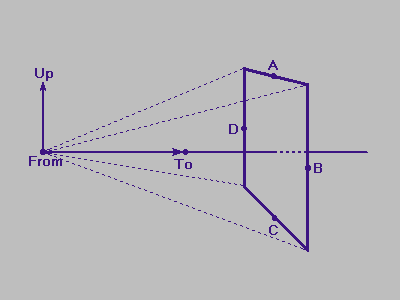Figure 3.3
The 3D Viewing Vector and Viewing Frustum

Refer to figure 3.3 for a diagram of the viewing parameters and viewing frustum in three dimensions. The angle from D to From to B is the horizontal viewing angle, and the angle from A to From to C is the vertical viewing angle.

To render a three-dimensional scene, we use these viewing parameters to project the scene to a two-dimensional rectangle, also known as the viewport. The viewport can be thought of as a window on the display screen between the eye (viewpoint) and the 3D scene. The scene is projected onto (or “through”) this viewport, which then contains a two-dimensional projection of the three-dimensional scene.

## 3.2 Viewing in Four-Space

To construct a viewing model for four dimensions, we extend the three-dimensional viewing model discussed in section 3.1 to four dimensions.

Three-dimensional viewing is the task of projecting the three-dimensional scene onto a two-dimensional rectangle. In the same manner, four-dimensional viewing is the process of projecting a 4D scene onto a 3D region, which can then be viewed with regular 3D rendering methods. The viewing parameters for the 4D to 3D projection are similar to those for 3D to 2D viewing.

As in the 4D viewing model, we need to define the from-point. This is conceptually the same as the 3D from-point, except that the 4D from-point resides in four-space. Likewise, the to-point is a 4D point that specifies the point of interest in the 4D scene.

The from-point and the to-point together define the line of sight for the 4D scene. The orientation of the image view is specified by the up-vector plus an additional vector called the over-vector. The over-vector accounts for the additional degree of freedom in four-space. Since the up-vector and over-vector specify the orientation of the viewer, the up-vector, over-vector and line of sight must all be linearly independent.

The viewing-angle is defined as for three-dimensional viewing, and is used to size one side of the projection-parallelepiped; the other two sides are sized to fit the dimensions of the projection-parallelepiped. For this work, all three dimensions of the projection parallelepiped are equal, so all three viewing angles are the same.

Figure 3.4 shows the projection of a 4D viewing frustum.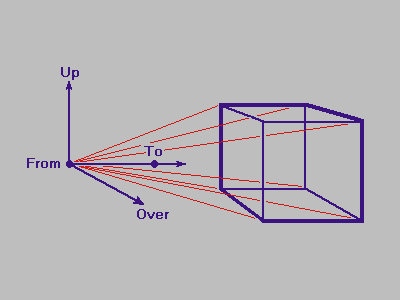Figure 3.4
The 4D Viewing Vectors and Viewing Frustum

# Chapter 4 Wireframe Display of Four Dimensional Objects

## 4.1 High-Level Overview of 4D to 2D Projection

Projection from four-space to a two-space region involves an additional projection compared to the usual display of three-dimensional wireframe data. Both the 3D projection and the additional 4D projection can be governed by independent sets of viewing parameters.

The first step of the 4D wireframe display process is to project the 4D vertices from four-space to an intermediate three-dimensional region. This projection uses the four dimensional viewing parameters discussed in section 3.2, and can be either a perspective projection or a parallel projection.

The next step is to take the projected vertices (now in three-space) and project them once more to the 2D viewport rectangle. This projection is determined by the three dimensional viewing parameters presented in section 3.1, and can also be either parallel or perspective. Once the vertices have been projected to screen coordinates, each edge of the wireframe is displayed.

## 4.2 Description of 3D to 2D Projection

There are several methods of projecting three-space points to a two-dimensional viewport. The method used and extended for this research is found in [Foley 87], and involves a vector subtraction and a multiplication between a 3-vector and a 3×3 matrix for each projected point.

The first step in projecting a 3D point is to convert its absolute “world” coordinates to viewer-relative “eye” coordinates. In the left-handed eye coordinate system, the eye-point is at the origin, the line-of-sight corresponds to the Z axis, the up-vector corresponds to the Y axis, and the X axis is orthogonal to the resulting Y and Z axes. Refer to figure 4.1 for a diagram of the eye-coordinate system.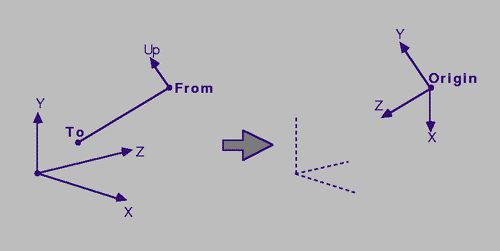Figure 4.1
3D Eye Coordinates

To convert a 3D point to 3D eye coordinates, one must first establish the vector from the eye-coordinate origin to the point by subtracting the from-point from the 3D vertex. Then the vector difference is rotated so that the to-point lies on the Z axis of the eye-coordinate system, and the up-vector lies on the Y axis. This is accomplished by multiplying the vector difference by the transformation matrix. The 3×3 transformation matrix has column vectors A, B and C, where A, B and C correspond to the X, Y, and Z axes in eye coordinates, respectively. The equations for these vectors are

To − From C = ⎯⎯⎯⎯⎯⎯⎯⎯⎯⎯⎯⎯⎯⎯, ‖ To − From ‖ Up ⨯ C A = ⎯⎯⎯⎯⎯⎯⎯⎯⎯⎯⎯, and ‖ Up ⨯ C ‖ B = C ⨯ A,

where To is the to-point, From is the from-point, Up is the up-vector, and the original world coordinates are supplied in the left-handed coordinate system. For the right-handed coordinate system, the cross product order for column vectors A and B would be reversed.

The procedure for computing the transformation matrix is given in the following algorithm. Note that Norm3(V) returns the vector norm of the 3-vector parameter V, and Cross3(U,V,W) assigns to U the 3-vector cross product of the parameter vectors V and W.

// The parameters a, b and c are the resulting transformation matrix // column vectors. void Calc3Matrix ( Point3 From, // Looking From This Point Point3 To, // Point of Interest Vector3 Up, // Direction Up Vector3 *Va, // Return Vector A Vector3 *Vb, // Return Vector B Vector3 *Vc) // Return Vector C { // Get the normalized Vc column-vector. VecSub (Vc, To, From); norm = Norm3 (Vc); if (norm == 0) Error ("To point and From point are the same."); VecScale (Vc, 1/norm); // Calculate the normalized Va column-vector. VecCross3 (Va, Vc, Up3); norm = Norm3 (Va); if (norm == 0) Error ("Up is parallel to the line of sight."); VecScale (Va, 1/norm); // Calculate the Vb column-vector. Cross3 (Vb, Va, Vc); }

Once the A, B and C vectors (corresponding to Va, Vb and Vc in the pseudo-code above) are calculated, all 3D points can be transformed from 3D world coordinates to 3D eye coordinates as follows:

⎡ Ax Bx Cx ⎤ P′ = [(Px−Fx) (Py−Fy) (Pz−Fz)] ⎢ Ay By Cy ⎢ Az Bz Cz

where Fx, Fy and Fz are the X, Y and Z coordinates of the from-point, P is the original data point in 3D world coordinates, and P′ is the transformed data point in eye coordinates.

We can now use the resulting 3D eye coordinates to project the 3D points to a two-dimensional rectangle. What we want is a projection that maps 3D points that lie in the 3D viewing frustum to the [−1,+1] × [−1,+1] rectangle. This rectangle will later be mapped to the viewport on the display device.

The projection from three-space to the 2D rectangle can be either a parallel projection or a perspective projection.

Parallel projection maps objects to the viewport in such a way that distant objects appear the same size as near objects. This is the effect that you'd get if the eye-point was infinitely far away from the object to be viewed. In the simple case where the projection plane is parallel to the XY plane, parallel projection can be achieved by dropping the Z coordinate (this is the case for eye coordinates). Scaling the projection to fit the [−1,+1] x [−1,+1] rectangle makes it easy to project the image to the viewport.

Perspective projection is the more natural of the two projections. With perspective projection, objects that are far away appear smaller than objects that are near. In the simple case, perspective projection is achieved by dividing by the Z coordinate. Perspective projection should map all data points that lie in the viewing frustum to the [−1,+1] × [−1,+1] rectangle.

When using parallel projection, the equation of the data point's normalized screen coordinates (from eye coordinates) is given by the following pair of equations:

P′x P′y Tx = ⎯⎯⎯⎯ and Ty = ⎯⎯⎯⎯, R3 R3 [4.2a]

where P′ is the 3D point in eye coordinates, R3 is the radius of the set of 3D points centered at the 3D to-point, and T is the 2D parallel projection of P′ to the [−1,+1] × [−1,+1] rectangle. Dividing by R3 ensures that the parallel projection fills the viewport as much as possible without extending past the viewport boundaries.

For the perspective projection of point P′, consider figure 4.2.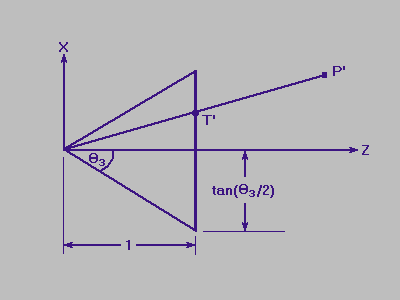Figure 4.2
3D Perspective Projection in Eye Coordinates

To calculate the perspective projection of point P′, we need to project the point to the viewplane and then to normalize the values so that points on the Z axis are projected to X (or Y) = 0, and so that the values of X (or Y) range from −1 to +1. The X axis value from figure 4.2 is calculated by noting that P′x/P′z = T′x/T′z. We can let T′z = 1 if the viewing angle is still preserved. Thus, T′x = P′x/P′z. To normalize T′x, note that the maximum possible value of T′x on the viewing plane occurs at T′x/T′z = tan(θ₃/2), or T′x = tan(θ₃/2). Thus, the equations for the normalized perspective projection T are given by

P′x P′y Tx = ⎯⎯⎯⎯⎯⎯⎯⎯⎯⎯⎯⎯⎯⎯ and Ty = ⎯⎯⎯⎯⎯⎯⎯⎯⎯⎯⎯⎯⎯⎯ P′z tan(θ₃/2) P′z tan(θ₃/2) [4.2b]

where θ₃ is the 3D viewing angle and T is the normalized perspective projection of P′ to the [−1,+1] × [−1,+1] rectangle. Note that in the equations presented in this chapter, the viewport is assumed to be square, so the viewing angle for the horizontal plane and the viewing angle for the vertical plane are the same. This assumption will also be held and extended for the 4D to 3D projection covered later.

Now that we have the points in the [−1,+1] × [−1,+1] rectangle, we'll need to map them to the viewport on the display device. This viewport is specified by the parameters

Cx, Cy
The viewport center, in screen coordinates, and
Lx, Ly
The horizonal & vertical length of the viewport, in screen coordinates.

Given these viewport parameters, the mapping of the point T in the [−1,+1] × [−1,+1] rectangle to the display device viewport is given by the following equations: Lx Ly Sx = Cx + ⎯⎯⎯Tx, and Sy = Cy + ⎯⎯⎯Ty. 2 2 [4.2c]

Putting this all together, we get the following algorithm. Note that the function Dot3(U,V) returns the dot product of the two 3-vector parameters U and V.

int NumVerts; // Number of 3D Vertices double Radius; // Radius of Vertices About the To3 Point Vector3 Va,Vb,Vc; // Viewing-Transformation Column Vectors Radians Vangle; // 3D Viewing Angle Vertex3 *VertList; // The Set of 3D Vertices void ProjectToScreen ( float Cx, float Cy, float Lx, float Ly, ProjectionType proj_type) { double S, T; // Divisor Values Vector3 V; // Scratch Vector if (proj_type == PARALLEL) S = 1 / Radius; else T = 1 / tan (Vangle / 2); for (i=0; i < NumVerts; ++i) { V = VertList[i].Position - From; if (proj_type == PERSPECTIVE) S = T / Dot3 (V, Vc); VertList[i].Screen[x] = Cx + (Lx * S * Dot3 (V, Va)); VertList[i].Screen[y] = Cy + (Ly * S * Dot3 (V, Vb)); } }

## 4.3 Description of 4D to 3D Projection

In this section, we extend the ideas and equations presented in section 4.2 to cover the projection of points from four-space to the intermediate 3D region.

It is possible to combine the 4D to 3D and 3D to 2D projections into a single step, but this approach lacks the flexibility of the following two-step approach. The two-step approach allows the user to independently specify viewing parameters for each projection, and to view the 3D projection from different angles while maintaining a constant 4D view.

Since the 4D to 2D projection takes place in two discrete steps, we'll need to specify an intermediate 3D region for the projection to 3D coordinates. For this research, the unit cube (edge length two) centered at the origin, with vertices <±1, ±1, ±1> was chosen as the intermediate region.

As in the 3D to 2D projections, the 4D data points can be projected to 3D space with either a perspective projection or a parallel projection. Neither of these projections are more “intuitive” than the other, but a perspective projection will yield smaller 3D line segments for edges that are farther from the 4D viewpoint. As an example of the differences between these projections, see section 4.6.

Changing the 3D projection type between perspective and parallel projection does not produce as dramatic (or puzzling) a change as for the 4D projection. However, switching back and forth can also provide a bit more understanding of the 4D-projected object.

The projection from 4D to 3D needs to be clipped at a minimum against the W=0 eye-coordinate plane. If both vertices have negative W eye-coordinate components, the edge should not be displayed. If both vertices have non-negative W components, then the edge can be displayed normally. If only one of the two vertices of a given edge has a negative W component, then the edge needs to be clipped to the W plane. This can be done by finding the intersection of the edge with the W plane and setting the vertex with the negative W component to the intersection point.

Since the 4D edges are projected to an arbitrary 3D region, it is not critical that they be clipped against the 4D viewing frustum. Edges that lie outside of the viewing frustum will lie outside the 3D region.

The first step of the 4D to 3D projection is to transform the vertices from their 4D world coordinates to the 4D eye coordinates. Refer to figure 4.3 for an illustration of the 4D eye coordinates.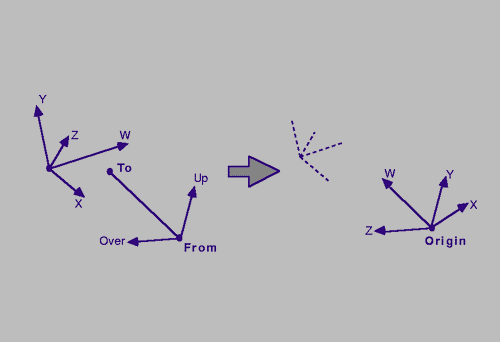Figure 4.3
4D Eye Coordinates

As in the 3D to 2D projection, this transformation is accomplished with a transformation matrix. The 4D viewing transformation matrix is composed of the column vectors A, B, C and D, which correspond to the X, Y, Z and W eye-coordinate axes, respectively. The equations for these column vectors are

To − From D = ⎯⎯⎯⎯⎯⎯⎯⎯⎯⎯⎯⎯⎯⎯, ‖ To − From ‖ X₄(Up,Over,D) A = ⎯⎯⎯⎯⎯⎯⎯⎯⎯⎯⎯⎯⎯⎯⎯⎯⎯⎯, ‖ X₄(Up,Over,D) ‖ X₄(Over,D,A) B = ⎯⎯⎯⎯⎯⎯⎯⎯⎯⎯⎯⎯⎯⎯⎯⎯⎯, and ‖ X₄(Over,D,A) ‖ C = X₄(D,A,B)

where To is the to-point, From is the from-point, Up is the up-vector, and Over is the Over vector (all of which reside in four-space).

The routine to calculate the four-dimensional transformation matrix follows.

// Calc4Matrix calculates the four-dimensional viewing transformation // matrix and places the resulting 4×4 matrix column vectors in // Wa, Wb, Wc, and Wd. Point4 From, To; // 4D From and To Points Vector4 Up, Over; // 4D Up and Over Vectors void Calc4Matrix ( Vector4 *Wa, Vector4 *Wb, Vector4 *Wc, Vector4 *Wd) { double norm; // Vector Norm // Get the normalized Wd column-vector. VecSub (Wd, To, From); norm = Norm4 (*Wd); if (norm == 0) Error ("To point and From point are the same."); VecScale (Wd, 1/norm); // Calculate the normalized Wa column-vector. Cross4 (Wa, Up,Over,*Wd); norm = Norm4 (Wa); if (norm == 0) Error ("Invalid Up vector."); VecScale (Wa, 1/norm); // Calculate the normalized Wb column-vector. Cross4 (Wb, Over, *Wd, *Wa); norm = Norm4 (Wb); if (norm == 0) Error ("Invalid Over vector."); VecScale (Wb, 1/norm); // Calculate the Wc column-vector. Cross4 (Wc, *Wd, *Wa, *Wb); }

The 4×4 matrix composed of these column vectors transforms the 4D world coordinates to 4D eye coordinates. The full transformation is given by the following product:

⎡ Ax Bx Cx Dx ⎤ V′ = [(Vx−Fx) (Vy−Fy) (Vz−Fz) (Vw−Fw)] ⎢ Ay By Cy Dy ⎢ Az Bz Cz Dz ⎢ Aw Bw Cw Dw ⎦,

where Fx, Fy, Fz and Fw are the coordinates of the from-point and Vx, Vy, Vz and Vw are the 4D world coordinates of the vertex. This equation yields the 4D eye-coordinates of the vertex: V′.

Now that the vertices have been transformed from 4D world coordinates to 4D eye coordinates, we can project them to the normalized [−1,+1] × [−1,+1] × [−1,+1] region in three-space. As for the 3D to 2D case, this projection can be either parallel or perspective. The equations for these projections are extensions of equations 4.2a and 4.2b.

The equations for parallel 4D to 3D projection are extended from equation 4.2a by one coordinate:

V′x V′y V′z Qx = ⎯⎯⎯, Qy = ⎯⎯⎯, and Qz = ⎯⎯⎯, R4 R4 R4 [4.3a]

where R4 is the radius of the set of 4D vertices about the to-point. Dividing by this radius ensures that the vertices are projected to fill the intermediate region as much as possible without extending past the boundaries.

In equation 4.2b, the X and Y eye coordinates are divided by the Z eye coordinate to yield the perspective projection. In the 4D to 3D perspective projection, this “depth” is similarly achieved by dividing by the W eye coordinate (which corresponds to the four-dimensional line-of-sight).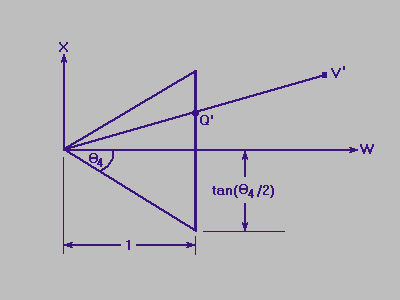Figure 4.4
4D Perspective Projection in Eye Coordinates

Figure 4.4 contains a diagram of the 4D normalized perspective projection. The derivation of the normalized 4D perspective projection follows the same reasoning as for the 3D normalized perspective projection. The equations are

V′x V′y V′z T = tan(θ₄/2), Qx = ⎯⎯⎯⎯⎯⎯, Qy = ⎯⎯⎯⎯⎯⎯, and Qz = ⎯⎯⎯⎯⎯⎯. V′w T V′w T V′w T [4.3b]

where θ₄ is the 4D viewing angle. These equations yield values in the range of [−1,+1] for vertices that lie in the 4D viewing frustum.

Mapping the projected points to a viewbox in three-space can be accomplished in the same manner that we mapped normalized 2D coordinates to the 2D viewport. Given the viewbox parameters

Bx, By, Bz
The center of the viewbox region, and

Dx, Dy, Dz
The length of the viewbox sides,

we can map the normalized 3D coordinates to the viewbox with the following equations:

Dx Dy Dz Px = Bx + ⎯⎯⎯ Qx, Py = By + ⎯⎯⎯ Qy, and Pz = Bz + ⎯⎯⎯ Qz. 2 2 2 [4.3c]

As mentioned earlier, the intermediate 3D region used in this research is the cube with vertices <±1, ±1, ±1>, centered at the three-space origin. For this particular region, Bx = By = Bz = 0, and Lx = Ly = Lz = 2, so the simplified equations are

V′x V′y V′z Px = ⎯⎯⎯⎯, Py = ⎯⎯⎯⎯, and Pz = ⎯⎯⎯⎯. R4 R4 R4

for 4D to 3D parallel projection, and

T = tan (θ₄/2) V′x V′y V′z Px = ⎯⎯⎯⎯⎯⎯, Py = ⎯⎯⎯⎯⎯⎯, and Pz = ⎯⎯⎯⎯⎯⎯. V′w T V′w T V′w T

for 4D to 3D perspective projection.

The routine to project the four-dimensional vertices to the three-dimensional region is given by the following algorithm (the algorithm presented here does not perform any type of 4D clipping):

double Radius; // Radius of the 4D Vertices int NumVerts; // Number of Vertices Radians Vangle; // 4D Viewing Angle Point4 *VertList; // Vertex Array Vector4 Wa,Wb,Wc,Wd; // 4D Transformation Matrix Column Vectors void ProjectTo3D (ProjectionType proj_type) { double S,T; // Divisor Values Vector4 V; // Scratch Vector if (proj_type == PARALLEL) S = 1 / Radius; else T = 1 / tan (Vangle / 2); for (i=0; i < NumVerts; ++i) { VecSub (V, VertList[i].Position,From); if (proj_type == PERSPECTIVE) S = T / Dot4 (V, Wd); VertList[i].Position = S * Dot4 (V, Wa); VertList[i].Position = S * Dot4 (V, Wb); VertList[i].Position = S * Dot4 (V, Wc); } }

## 4.4 Rotations of 4D Wireframes

Rotation of the 4D wireframe is a tremendous aid in understanding the fundamental structure of the displayed object. This rotation is best done by tying the rotation input to mouse movement. The wireframe program written for this research uses the horizontal movement of the mouse only. Restricting the rotation input to a single plane helps only somewhat for three-space rotation, but greatly helps with four-space rotations, where it's more difficult to figure out how to “undo” a particular pair of rotations of the four-space viewpoint.

Rotating the view of the object can be accomplished by rotating the viewpoint, rather than rotating each of the object vertices. This way, it isn't necessary to rotate all of the wireframe vertices; you only have to rotate the viewpoint. For rotation in three-space, use the regular 3D rotation matrices, and for rotating in four-space, use the rotation matrices presented in section 2.2. Another way to describe this is to say that the 3D (or 4D) from-point is moved over a three- (or four-) sphere.

When rotating the three-space view, you don't need to recompute the 4D to 3D projections; it's more efficient to save the projected 3D vertices and to recompute only the 3D to screen projections. The main steps for rotating the 3D viewpoint are:

1. Rotate the 3D from-point about the 3D to-point in some plane.
2. Recalculate the 3D viewing transformation matrix.
3. Project all 3D points to viewport coordinates.
4. Display each wireframe edge.

When rotating the four-space viewpoint, you also need to recompute the 4D to 3D projection. The main steps for rotating the 4D viewpoint are:

1. Rotate the 4D viewpoint about the 4D to-point in some plane.
2. Recalculate the 4D viewing transformation matrix.
3. Project all 4D points to the 3D cube space.
4. Project all 3D points to viewport coordinates.
5. Display each wireframe edge.

## 4.5 User Interaction and Visualization Aids

User interaction for the wireframe program should include several options to allow the user to experiment with the displayed object. One of the most important of these options is the interactive rotation of the object mentioned above, but there are several other options that increase the understanding of the wireframe object.

The user should be able to switch between perspective and parallel projection of the wireframe for both the 4D to 3D projection and the 3D to viewport projection. Switching from parallel projection to perspective projection sometimes gives the user a better idea of the object's perspective.

Another aid is the display of both the 3D and the 4D coordinate axes. This display aids the user in orienting the object with the 3D or 4D world, and also helps the user to choose the desired object rotation; this is especially helpful when trying to choose four-space rotations.

Displaying the edges of the <±1, ±1, ±1> cube (the intermediate three-dimensional projection space) along with the object helps the user to select the proper 3D and 4D viewing parameters in order to best fill the intermediate 3D cube and 2D viewport. It also helps the user to identify rotations in four-space versus rotations in three-space without looking away from the object display.

Finally, a useful four-dimensional visual aid is the depthcueing of the wireframe according to the four-dimensional depth of each vertex. In normal three-dimensional depthcueing, the Z eye-coordinate is used to assign an intensity to the vertex. Edges are then shaded by linearly interpolating the intensities of the two endpoint vertices. Typically, vertices that are farther from the viewpoint are rendered with a lower intensity, and vertices closer to the viewpoint are rendered with greater intensity, so edges dim in intensity as they extend away from the viewer.

This analogy extends quite nicely to four-dimensional wireframes; the “depth” of a vertex is simply the 4D W eye-coordinate. As an example, when the four-cube is rendered with 4D depthcueing, the “inner” cube is shaded with a lower intensity than the “outer” cube, since it is farther away in four-dimensional space.

## 4.6 Example 4D Wireframe Images

In figures 4.5a through 4.5d, the hypercube with vertices of <±1, ±1, ±1, ±1> is rendered with 4D parallel & perspective and 3D parallel & perspective projections. The four-cube is displayed with the following viewing parameters:

```    From4  = <4, 0, 0, 0>
To4    = <0, 0, 0, 0>
Up4    = <0, 1, 0, 0>
Over4  = <0, 0, 1, 0>
theta4 = 45 degrees
From3  = <3.00, 0.99, 1.82>
To3    = <0, 0, 0>
Up3    = <0, 1, 0>
theta3 = 45 degrees
```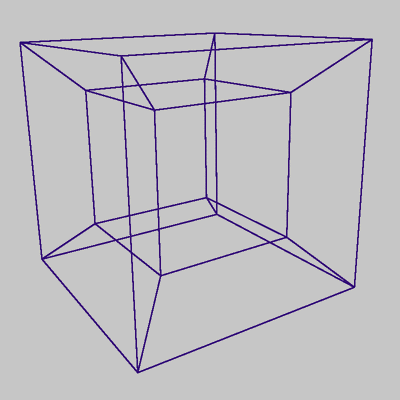Figure 4.5a:
4D Perspective and 3D Perspective Projection
[The 4-Cube with Various 4D and 3D Projections]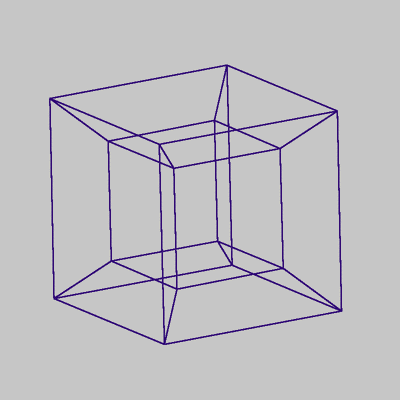Figure 4.5b:
4D Perspective and 3D Parallel Projection
[The 4-Cube with Various 4D and 3D Projections]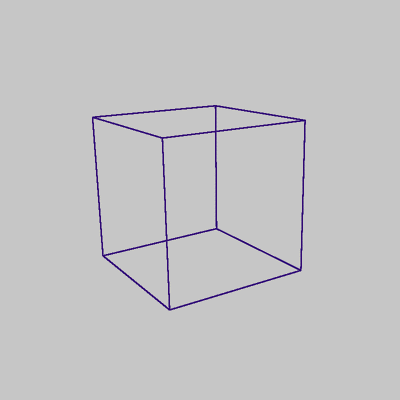Figure 4.5c:
4D Parallel and 3D Perspective Projection
[The 4-Cube with Various 4D and 3D Projections]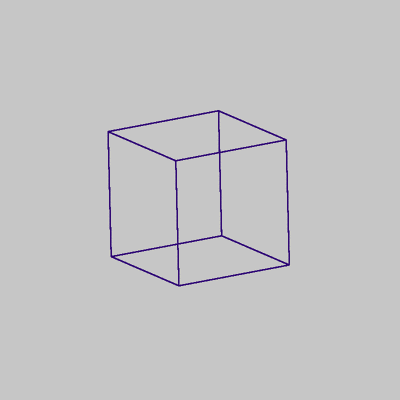Figure 4.5d:
4D Parallel and 3D Parallel Projection
[The 4-Cube with Various 4D and 3D Projections]

In figure 4.5a, the “inner” cube is actually farther away in four-space than the “outer” cube, and hence appears smaller in the resulting projection. You can think of the larger cube as the “front face” of the four-cube, and the the smaller cube as the “rear face” of the four-cube. When rotating the four-cube in the proper plane, the rear face gradually swings to the front, and the front face gradually swings to the rear. In doing this, the cube appears to turn itself inside out, so that the originally smaller cube engulfs the previously larger cube.

In figure 4.5c, the four-cube is displayed with 4D parallel projection and 3D perspective projection. Because of the parallel projection from 4D, the “rear face” and “front face” are displayed as the same size, so the parallel projection from this point in four-space looks like two identically-sized three-cubes superimposed over each other.

Figures 4.6a through 4.6d are similar to figures 4.5a through 4.5d, except that the four-dimensional viewpoint has changed. For these views, the four-dimensional viewing parameters are:

```    From4  = <2.83, 2.83, .01, 0>
To4    = <0, 0, 0, 0>
Up4    = <-.71, .71, 0, 0>
Over4  = <0, 0, 1, .02>
theta4 = 45 degrees
From3  = <3.29, .68, 1.4>
To3    = <0, 0, 0>
Up3    = <.08, 1, .04>
theta3 = 45 degrees
```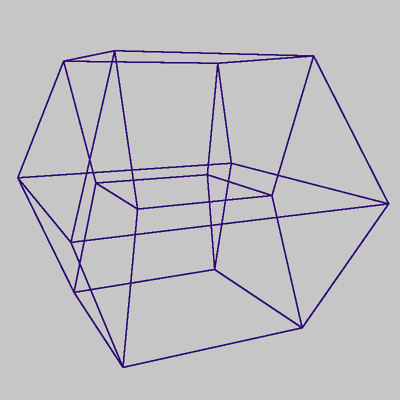Figure 4.6a:
4D Perspective and 3D Perspective Projection
[Another View of The 4-Cube with Various 4D and 3D Projections]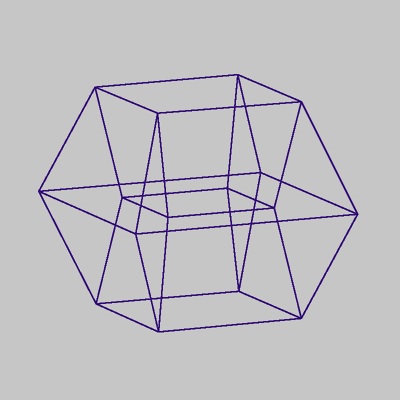Figure 4.6b:
4D Perspective and 3D Parallel Projection
[Another View of The 4-Cube with Various 4D and 3D Projections]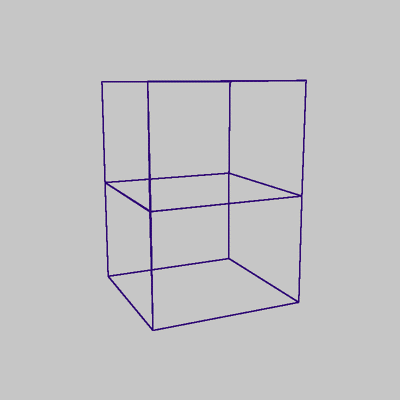Figure 4.6c:
4D Parallel and 3D Perspective Projection
[Another View of The 4-Cube with Various 4D and 3D Projections]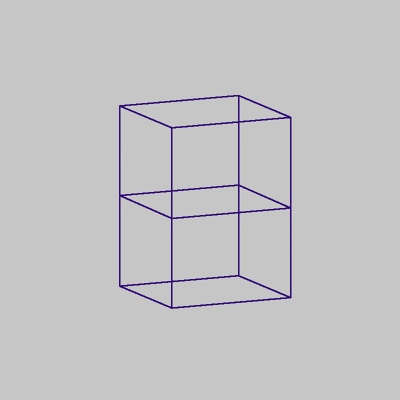Figure 4.6d:
4D Parallel and 3D Parallel Projection
[Another View of The 4-Cube with Various 4D and 3D Projections]

This vantage point occurs one eighth of the way though a complete four-dimensional rotation. See figure 4.7a for an illustration of this rotation.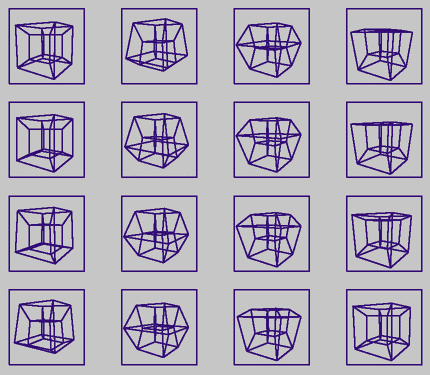Figure 4.7a:
4D Perspective and 3D Perspective Projection
4D Rotation of the 4-Cube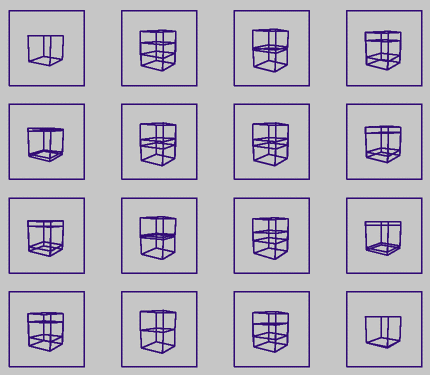Figure 4.7b:
4D Parallel and 3D Perspective Projection
4D Rotation of the 4-Cube

Figure 4.7a shows the sequence of one fourth of a four-dimensional rotation of the hypercube (read the sequence from top to bottom, left to right) with 4D and 3D perspective projection. Figure 4.7b shows the same sequence with 4D parallel and 3D perspective projection.

In figure 4.9, the dual of the four-cube is rendered with all edges rendered the same color. The dual of the four-cube is the wireframe of convex hull of the face centers of the four-cube. In other words, the convex hull of the points <±1, 0, 0, 0>, <0, ±1, 0, 0>, <0, 0, ±1, 0>, and <0, 0, 0, ±1>. One could also think of it as the four-dimensional analog of the three-dimensional octahedron.

Figures 4.8 through 4.13 illustrate the differences in single edge-color rendering, multiple edge-color rendering, and depth-cued edge rendering. Even with interactive manipulation of the four-dimensional wireframe, single edge-color rendering yields an image that is difficult to interpret. Assigning different colors to the edges greatly aids the user in identifying sub-structures of the four-dimensional wireframe, and serves as a structural reference when rotating the object. Depth-cueing the edges gives a spatial sense of the object, but loses the structural cues.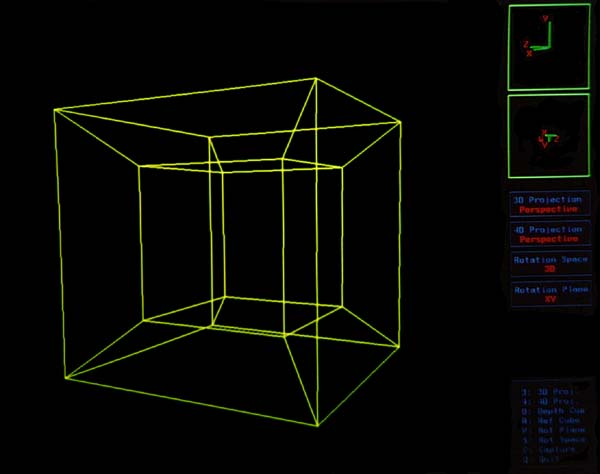Figure 4.8
The 4-Cube With All Edges Rendered in One Color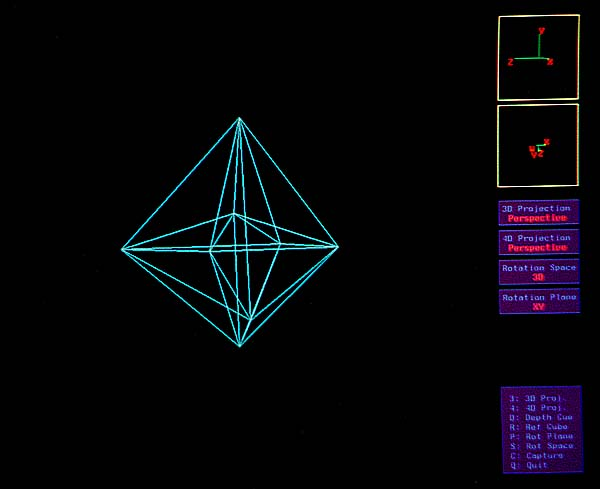Figure 4.9
The Dual of The 4-Cube With All Edges Rendered in One Color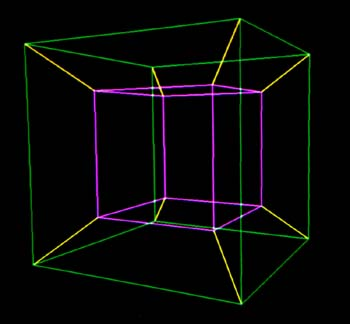Figure 4.10
The 4-Cube Rendered With Multiple Edge Colors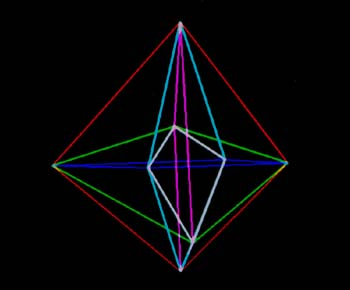Figure 4.11
The Dual of The 4-Cube Rendered With Multiple Edge Colors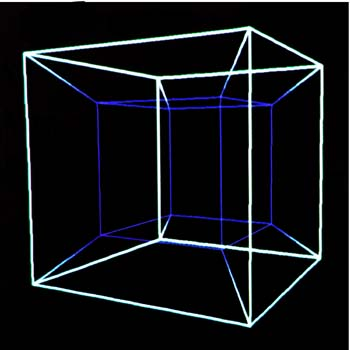Figure 4.12
The 4-Cube Rendered With Depth-Cueing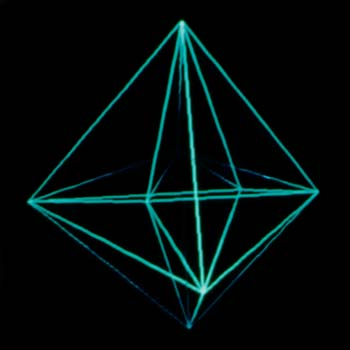Figure 4.13
The Dual of The 4-Cube Rendered With Depth-Cueing

Finally, figures 4.14 and 4.15 show generalized curves across the surface of a four-sphere. The curve in figure 4.15 is given with poor uniform parameterization which yields the two “kinks” that are visible in the 4D image. For more information on these particular curves and the choices of parameterization, refer to [Chen 90].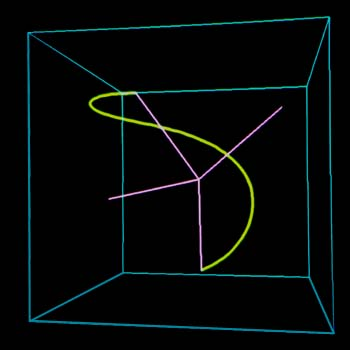Figure 4.14
A 4D Curve on a 4-Sphere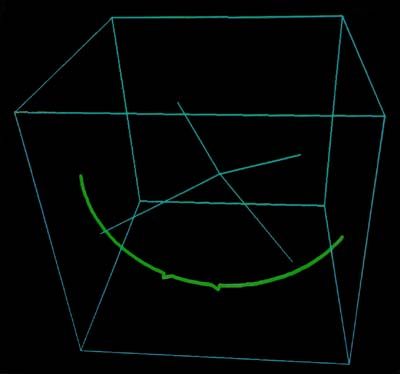Figure 4.15
A 4D Curve on a 4-Sphere with Poor Parameterization

# Chapter 5 Raytracing in Four Dimensions

## 5.1 General Description of the Raytracing Algorithm

Wireframe rendering has several advantages over other rendering methods, including simplicity of representation, speed of display, and ease of implementation. However, it cannot render solid objects, or objects that obscure one another. In addition, it cannot model other aspects of light propagation, such as shadows and reflections, which aid the user in understanding a given scene.

Other rendering techniques exist that solve the hidden surface problem and shadows by representing the objects with a tessellated mesh of polygons. These algorithms map the polygons to the viewport in a particular order to solve for hidden surfaces. These algorithms must also handle the cases of partially obscured polygons. However, these techniques are not easily extended to four-dimensional rendering. Instead of dealing only with planar polygons, the four-dimensional counterpart would have to deal with tessellating solids; thus, it would also have to properly handle intersecting solids with hidden volumes and solids that partially obscure one another. This is at best a difficult task in three-space; the four-space extension would be even more complex.

For these reasons, the raytracing algorithm was chosen to “realistically” render four-space scenes. Raytracing solves several rendering problems in a straight-forward manner, including hidden surfaces, shadows, reflection, and refraction. In addition, raytracing is not restricted to rendering polygonal meshes; it can handle any object that can be interrogated to find the intersection point of a given ray with the surface of the object. This property is especially nice for rendering four-dimensional objects, since many N-dimensional objects can be easily described with implicit equations.

Other benefits of raytracing extend quite easily to 4D. As in the 3D case, 4D raytracing handles simple shadows merely by checking to see which objects obscure each light source. Reflections and refractions are also easily generalized, particularly since the algorithms used to determine refracted and reflected rays use equivalent vector arithmetic.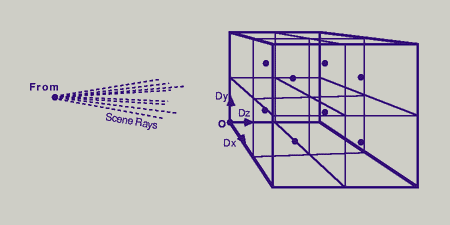Figure 5.1
A 2x2x2 4D Raytrace Grid

The main loop in the raytracing algorithm shoots rays from the viewpoint through a grid into the scene space. The grid is constructed so that each grid element represents a voxel of the resulting image (see figure 5.1 for an illustration of a 2×2×2 ray grid). As a ray is “fired” from the viewpoint through the grid, it gathers light information by back-propagation. In this way raytracing approximates the light rays that scatter throughout the scene and enter the eye by tracing the rays back from the viewpoint to the light sources.

The recursive nature of raytracing, coupled with the fact that every voxel is sampled, makes raytracing very time consuming. Fortunately, extending the raytracing algorithm to four dimensions does not necessarily incur an exponential increase in rendering time. However, finding some ray-object intersections does entail a significant increase in computation. For example, determining the intersection of a four-space ray with a four-space tetrahedron is much more expensive than computing the intersection of a three-space ray with a three-space triangle. This increase of complexity does not necessarily occur with all higher-order object intersections, though. The hypersphere, for example, can be intersected with essentially the same algorithm as for the three-sphere (although vector and point operations must handle an extra coordinate).

## 5.2 Generating the Four-Dimensional Ray Grid

The ray grid must be constructed so that each point on the grid corresponds to each pixel for 3D raytracing or voxel (volume element) for 4D raytracing. In four-dimensional raytracing, the grid is a three-dimensional parallelepiped spanned by three orthogonal vectors. Note that although in figure 5.1 it seems that a scene ray would pass through other voxels as it intersects each voxel center, scene rays do not lie in the same three-space (or hyperplane) as the ray grid. As a result, each scene ray intersects the ray grid only at the voxel centers.

The ray grid is constructed from the viewing parameters presented in section 3.2. These viewing parameters are the same as the viewing parameters used for the 4D wireframe viewer.

The viewpoint is the point of origin for the scene rays, so it must be outside of the ray grid. Since the to-point is the point of interest, it should be centered in the 4D ray grid.

Now that we have the center of the ray grid, we need to establish the basis vectors of this grid. Once we do that, we can index a particular voxel in the grid for the generation of scene rays.

The up-vector and over-vector are used to form two of the grid basis vectors (after proper scaling). Since the line of sight must be perpendicular to the ray grid, we can generate the third basis vector by forming the four-dimensional cross product of the line of sight with the up-vector and over-vector. Note that in four-space, a ray can pass through any point within the cube without intersecting any other point.

The grid basis vectors are computed as follows:

From − To S = ⎯⎯⎯⎯⎯⎯⎯⎯⎯⎯⎯⎯⎯ ‖From − To‖ cross4 (Over, Up, S) Bz = ⎯⎯⎯⎯⎯⎯⎯⎯⎯⎯⎯⎯⎯⎯⎯⎯⎯⎯⎯⎯⎯⎯⎯⎯ ‖cross4 (Over, Up, S)‖ cross4 (Bz, S, Over) By = ⎯⎯⎯⎯⎯⎯⎯⎯⎯⎯⎯⎯⎯⎯⎯⎯⎯⎯⎯⎯⎯⎯⎯⎯ ‖cross4 (Bz, S, Over)‖ Bx = cross4 (By, Bz, S)

At this point, S is the unit line-of-sight vector, and Bx, By & Bz are the unit basis vectors for the ray grid. What we need to do now is to to scale these vectors. There are two additional sets of parameters that govern the construction of the ray grid. These are the the number of voxels along each axis of the grid (the resolution of the ray grid), and the shape of each voxel (the aspect ratios). In addition, we need to incorporate the viewing angle.

The resolution of the grid cube is given by the parameters Rx, Ry and Rz, which specify the number of voxels along the width, height and depth of the cube, respectively. The aspect ratio of each voxel is given by the parameters Ax, Ay and Az. These parameters specify the width, height and depth of each voxel in arbitrary units (these numbers are used only in the ratio). For example, an aspect ratio of 1∶4∶9 specifies a voxel that is four times as high as it is wide, and that is 4/9ths as high as it is deep.

The ray grid is centered at the to-point; we use the viewing angle to determine the ray-grid size. As mentioned earlier, the viewing angle corresponds to the X axis. The other axes are sized according to the resolution and aspect ratios. Determining the proper scale of the grid X axis is easily done from the viewing angle

Lx = 2 ‖From−To‖ tan(θ₄/2)

where θ₄ is the 4D viewing angle, and Lx is the width of the ray grid. The other dimensions of the ray grid are determined by Lx, the aspect ratios, and the resolutions:

Ry Ay Rz Az Ly = Lx ⎯⎯ ⎯⎯, and Lz = Lx ⎯⎯ ⎯⎯ Rx Ax Rx Ax

Thus, Lx, Ly and Lz are the lengths of each edge of the ray grid, and the grid basis vectors are scaled with these lengths to yield

Gx = Lx Bx, Gy = Ly By, and Gz = Lz Bz.

The main ray loop will start at a corner of the ray grid and scan in X, Y and Z order, respectively. The origin of the grid (each basis vector zero) is given by

Gx + Gy + Gz O = To − ⎯⎯⎯⎯⎯⎯⎯⎯⎯⎯⎯⎯⎯⎯. 2

The incremental grid vectors are used to move from one grid voxel to another. They are computed by dividing the grid-length vectors by the respective resolution:

Gx Gy Gz Dx = ⎯⎯⎯⎯, Dy = ⎯⎯⎯⎯, Dz = ⎯⎯⎯⎯. Rx Ry Rz

Finally, the grid origin is offset by half a voxel, in order that the voxel centers are sampled.

Dx + Dy + Dz O = O + ⎯⎯⎯⎯⎯⎯⎯⎯⎯⎯⎯⎯⎯ 2

The main raytracing procedure looks like this:

Vector4 Dx,Dy,Dz; // Grid-Traversal Vectors Point4 From4; // 4D Viewpoint Point4 Origin; // Grid Origin Corner int Rx,Ry,Rz; // Grid Resolutions void FireRays (void) { int i,j,k; // Grid Traversal Indices Vector4 T; // Scratch Vector Ray4 ray; // 4D View Ray for (i=0; i < Rx; ++i) { for (j=0; j < Ry; ++j) { for (k=0; k < Rz; ++k) { T = Origin + i*Dx + j*Dy + k*Dz; VecCopy (ray.origin, From); VecSub (ray.direction, T,ray.origin); // Recursively fire sample rays into the scene. Raytrace (ray); } } } }

## 5.3 The General Raytrace Algorithm

Each ray is propagated throughout the scene in the following manner:

1. For each point in the ray grid, fire a ray from the viewpoint through the grid point.
2. Find the intersection of the ray with all objects in the scene. If the ray intersects no objects in the scene, assign the background color to it.
3. The intersection point closest to the “launch point” (starting with the viewpoint) is chosen, and the current color is determined by the ambient color of the intersected object.
4. The intersection point is the new launch-point. Rays are fired from the launch point to each of the light sources. If the ray does not intersect any other object first, the current point is then further illuminated by that light source to yield the diffuse and specular components of the object. This occurs for all light sources.
5. If the object has a reflective surface, then a ray is recursively reflected from the current point and gathers color information by going back to step two above.
6. If the object has a refractive surface, then a ray is recursively refracted from the current point and gathers color information by going back to step two above.
7. The color obtained by steps three through six is assigned to the voxel that corresponds to the current grid point.

## 5.4 Reflection and Refraction Rays

The reflection and refraction rays mentioned in the previous section are generated in the same way as they are for 3D raytracing, with the exception that the vector arithmetic is of four dimensions rather than three. Since reflection and refraction rays are confined to the plane containing the normal vector and the view vector, reflection and refraction rays are given by the following equations for raytracing in any dimension higher than one.

Refer to figure 5.2 for a diagram of the reflection ray.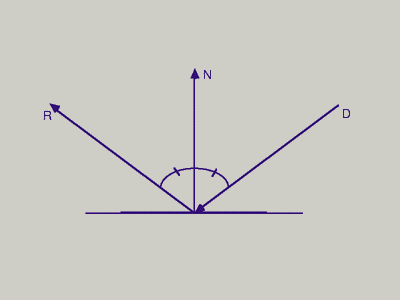Figure 5.2
Ray-Object Reflection

The equation of the reflection ray is given by is

R = D − 2(N·D)N

where R is the resulting reflection ray, D is the unit direction of the light ray towards the surface, and N is the unit vector normal to the surface. Refer to [Foley 87] for a derivation of the reflection equation.

The refraction ray T is given by

T = dC + (1−d)(−N) D C = ⎯⎯⎯⎯⎯⎯⎯⎯ ‖N·D‖ 1 d = ⎯⎯⎯⎯⎯⎯⎯⎯⎯⎯⎯⎯⎯⎯⎯⎯⎯⎯⎯⎯⎯⎯⎯⎯⎯⎯⎯⎯⎯⎯⎯⎯⎯⎯ sqrt(((r₁/r₂)² · ‖C‖²) − ‖C+N‖²)

where T is the refraction ray, D is the unit direction of the light ray towards the surface, N is the unit normal vector to the surface, r₁ is the index of refraction of the medium containing the light ray, and r₂ is the index of refraction of the object. Note that this equation does not yield a unit vector for T; T must be normalized after this equation. Refer to [Hill 90] for a derivation of this formula.

## 5.5 Illumination Calculations

The illumination equations for four-dimensional raytracing are the same as those for raytracing in three dimensions, although the underlying geometry is changed. A simple extended illumination equation is as follows:

N ⎲ I = Ia·Ka + ⎳ IL(Kd·cos(θ) + Ks·cosⁿ(α)) + Ks·Ir + Kt·It. L=1

The values used in this equation are

 Ia [RGB] Global ambient light. IL [RGB] Light contributed by light L. Ir [RGB] Light contributed by reflection. It [RGB] Light contributed by transmission (refraction). Ka [RGB] Object ambient color. Kd [RGB] Object diffuse color. Ks [RGB] Object reflection color. Kt [RGB] Object transparent color. n [Real] Phong specular factor. N [Integer] Number of light sources.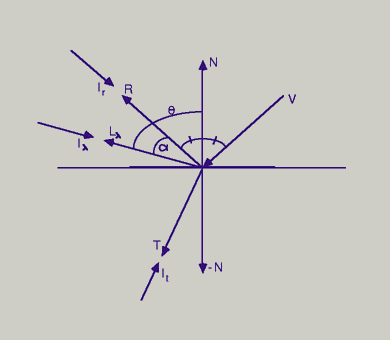Figure 5.3
Components of Illumination

Refer to figure 5.3 for a diagram of the illumination vectors and components. The angle theta between the surface normal vector and the light direction vector determines the amount of diffuse illumination at the surface point. The angle α is the angle between the reflected light vector and the viewing vector, and determines the the amount of specular illumination at the surface point. These angles are given by the following formulas.

cos(θ) = N·Lλ cos(α) = R·Lλ

If cos(θ) is negative, then there is no diffuse or specular illumination at the surface point. If cos(θ) is non-negative and cos(α) is negative, then the surface point has diffuse illumination but no specular illumination.

In the summation loop, a ray is fired from the surface point to each light source in the scene. If this shadow ray intersects any other object before the light source, then the contribution from that light source is zero; Iλ for light source λ is set to zero. If no object blocks the light source, then Iλ is used according to the type of light source.

The raytracer developed for this research implements both point and directional light sources. For directional light sources, the vector Lλ is constant for all points in the scene. For point light sources, Lλ is calculated by subtracting the point light source location from the surface point. Both of these light sources are assigned a color value (Iλ).

## 5.6 Intersection Algorithms

The fundamental objects implemented in the 4D raytracer include hyperspheres, tetrahedra and parallelepipeds. The intersection algorithms for each of these objects takes a pointer to the object to be tested plus the origin and unit direction of the ray. If the ray does not intersect the object, the function returns false. If the ray hits the object, the function returns the intersection point and the surface normal at the intersection point.

Some objects, such as hyperspheres, can have zero, one or two intersection points. More complex objects may well have many more intersection points. The intersection functions must return the intersection point closest to the ray origin (since other intersection points would be obscured by the nearest one.)

### 5.6.1 Ray-Hypersphere Intersection

The hypersphere is one of the simplest four-dimensional objects, just as the three-sphere is among the simplest objects in 3D raytracers. Like the three-sphere, the four-sphere is specified by a center point and a radius.

The implicit equation of the four-sphere is

(Sx − Cx)² + (Sy − Cy)² + (Sz − Cz)² + (Sw − Cw)² − r² = 0

where r is the radius of the four-sphere, C is the center of the sphere, and S is a point on the surface of the sphere.

Obtaining the normal vector from the intersection point is a trivial matter, since the surface normal of a sphere always passes through the center. Hence, for an intersection point I, the surface normal at I is given by N = I − C.

Calculating the intersection of a ray with the sphere is also fairly straight-forward. Given a ray defined by the equation r = P + tD, where P is the ray origin, D is the unit ray direction vector, and t is a parametric variable, we can find the intersection of the ray with a given hypersphere in the following manner:

(Cx−Sx)² + (Cy−Sy)² + (Cz−Sz)² + (Cw−Sw)² − r² = 0 ‖C − S‖² − r² = 0

Substitute the ray equation into the surface value to get

‖−(P + tD)‖² − r² = 0 ‖(C−P) − tD‖² − r² = 0 ‖V−tD‖² − r² = 0 where V = C−P (Vx−tDx)² + (Vy−tDy)² + (Vz−tDz)² + (Vw−tDw)² − r² = 0 t²(Dx² + Dy² + Dz² + Dw²) − 2t(VxDx + VyDy + VzDz + VwDw) + (Vx² + Vy² + Vz² + Vw²) − r² = 0

This simplifies to

t²(D·D) − 2t(V·D) + (V·V − r²) = 0.

Since D is a unit vector, this equation further simplifies to

t² − 2t(V·D) + (V·V − r²) = 0.

The quadratic formula x² − 2bx + c = 0 has roots b ± sqrt(b²−c). So, solving for t, we get

t = (V·D) ± sqrt((V·D)² − (V·V − r²)).

The intersection point is given by plugging the smallest non-negative solution for t into the ray equation. If there is no solution to this equation (e.g., the quantity under the square root is negative), then the ray does not intersect the hypersphere.

The pseudo-code for the ray-hypersphere intersection algorithm follows.

### 5.6.2 Ray-Tetrahedron Intersection

The tetrahedron is to the 4D raytracer what the triangle is to the 3D raytracer. Just as all 3D objects can be approximated by an appropriate mesh of tessellating triangles, 4D objects can be approximated with an appropriate mesh of tetrahedra. Of course, the tessellation of 4D objects is more difficult (e.g. how do you tessellate a hypersphere?), but it does allow for the approximation of a wide variety of objects.

In the fourth dimension, the tetrahedron is “flat”, i.e. it has a constant normal vector across its volume. Any vector embedded in the tetrahedron is perpendicular to the tetrahedron normal vector.

The tetrahedron is specified by four completely-connected vertices in four-space. A tetrahedron in which the four vertices are coplanar is a degenerate tetrahedron; it is analogous to a triangle in three-space with colinear vertices. The 4D raytracer should ignore degenerate tetrahedra as invisibly thin.

Since the tetrahedron normal is constant, pre-compute this vector and store it in the tetrahedron description before raytracing the scene. The normal is computed by finding three independent vectors on the tetrahedron and crossing them to compute the orthogonal normal vector.

B₁ = V₁ − V₀, B₂ = V₂ − V₀, B₃ = V₃ − V₀, and X4(B₁,B₂,B₃) N = ⎯⎯⎯⎯⎯⎯⎯⎯⎯⎯⎯⎯⎯⎯⎯⎯ ‖X4(B₁,B₂,B₃)‖

where V₀, V₁, V₂, and V₃ are the tetrahedron vertices and N is the unit normal vector.

Finding the intersection point of a ray and tetrahedron is much more difficult than for the hypersphere case. This is primarily because it requires the solution of a system of three equations and three unknowns to find the barycentric coordinates of the intersection point.

Once the barycentric coordinates of the intersection point are known, they can be used to determine if the point lies inside the tetrahedron, and also to interpolate vertex color or vertex normal vectors across the hyperface of the tetrahedron (Gouraud or Phong shading, respectively). For further reference on barycentric coordinates, refer to [Farin 88] and [Barnhill 84] (particularly the section on simplices and barycentric coordinates).

The method used to find the barycentric coordinates of the ray-hyperplane intersection with respect to the tetrahedron is an extension of the algorithm for computing barycentric coordinates of the ray-plane intersection with respect to the triangle, presented in [Glassner 90].

Again, the ray is specified by the equation P + tD, where P is the ray origin, D is the unit direction vector, and t is the ray parameter. For each point Q on the tetrahedron, Q·N is constant. Let d = −V₀·N. Thus, the hyperplane is defined by N·Q + d = 0, where the tetrahedron is embedded in this hyperplane.

First compute the ray-hyperplane intersection with t = −(d + N·P) / (N·D). If N·D is zero, then the ray is parallel to the embedding hyperplane; it does not intersect the tetrahedron. If t < 0, then the embedding hyperplane is behind the ray, so the ray does not intersect the tetrahedron.

Now compute the ray-hyperplane intersection with relation to the tetrahedron. The barycentric coordinates of the intersection point Q is given by the equation

___ ____ ____ ____ V₀Q = α · V₀V₁ + β · V₀V₂ + γ · V₀V₃. [5.5.2a]

The ray-hyperplane intersection point Q is inside the tetrahedron if α ≥ 0, β ≥ 0, γ ≥ 0, and α+β+γ ≤ 1.

Equation 5.5.2a can be rewritten as

⎡Qx − V0x⎤ ⎡V1x − V0x⎤ ⎡V2x − V0x⎤ ⎡V3x − V0x⎤ ⎢Qy − V0y⎥ = α ⎢V1y − V0y⎥ + β ⎢V2y − V0y⎥ + γ ⎢V3y − V0y⎥ ⎢Qz − V0z⎥ ⎢V1z − V0z⎥ ⎢V2z − V0z⎥ ⎢V3z − V0z⎥ ⎢Qw − V0w⎥ ⎢V1w − V0w⎥ ⎢V2w − V0w⎥ ⎢V3w − V0w⎥ ⎣ ⎦ ⎣ ⎦ ⎣ ⎦ ⎣ ⎦. [5.5.2b]

To simplify the solution for these coordinates, we project the tetrahedron to one of the four primary hyperplanes (XYZ, XYW, XZW or YZW). To make this projection as “large” as possible (to ensure that we don't “flatten” the tetrahedron by projecting it to a perpendicular hyperplane), find the dominant axis of the normal vector and use the hyperplane perpendicular to the dominant axis. In other words the normal to the major hyperplane is formed by replacing the normal coordinate that has the largest absolute value with zero. For example, given a normal vector of <3, 1, 7, 5>, the dominant axis is the third coordinate, and the hyperplane perpendicular to <3, 1, 0, 5> will yield the largest projection of the tetrahedron. Once again, since the normal vector is constant, the three non-dominant coordinates (X, Y, and W for the above example) should be stored for future reference. Refer to the intersection algorithm for an illustration of this.

The hyperplane equation is then reduced to three coordinates, i, j, and k (X, Y & W for the previous example), so equation [5.5.2b] is reduced to

⎡Qi − V0i⎤ ⎡V1i − V0i⎤ ⎡V2i − V0i⎤ ⎡V3i − V0i⎤ ⎢Qj − V0j⎥ = α ⎢V1j − V0j⎥ + β ⎢V2j − V0j⎥ + γ ⎢V3j − V0j⎥. ⎢Qk − V0k⎥ ⎢V1k − V0k⎥ ⎢V2k − V0k⎥ ⎢V3k − V0k⎥ ⎣ ⎦ ⎣ ⎦ ⎣ ⎦ ⎣ [5.5.2c]

Now find α, β, and γ by solving the system of three equations and three unknowns; these are the barycentric coordinates of the intersection point Q relative to the tetrahedron. The fourth barycentric coordinate is given by (1 − α − β − γ).

In order for the tetrahedron to contain the ray-hyperplane intersection point, the following equations must be met:

α ≥ 0, β ≥ 0, γ ≥ 0, and (α + β + γ) ≤ 1.

If any of the barycentric coordinates are less than zero, or if the barycentric coordinates sum to greater than one, then the ray does not intersect the tetrahedron.

Once α, β and γ are known for the point of intersection, the ray-hyperplane intersection point Q can be found by the following equation: Q = (1−α−β−γ)V₀ + αV₁ + βV₂ + γV₃.

The following pseudo-code implements the ray-tetrahedron intersection algorithm. function HitTet: Boolean ( ray: Ray4, tet: Tetrahedron, intersect: Point4, normal: Vector4 ) A11,A12,A13: Real Equation System Matrix Values A21,A22,A23: Real A31,A32,A33: Real b1, b2, b3 : Real Equation System Results rayt: Real Ray Parameter Value for Intersection x1, x2, x3 : Real Equation System Solution Compute the intersection of the ray with the hyperplane containing the tetrahedron. rayt <- Dot4 (tet.normal,ray.direction) if rayt < 0 return false rayt <- - (tet.HPlaneConst + Dot4 (tet->normal,ray.origin)) / rayt if rayt < 0 return false Calculate the intersection point of the ray and embedding hyperplane. intersect <- ray.origin + (rayt * ray.direction) Calculate the equation result values. Note that the dominant axes are precomputed and stored in tet.axis1, tet.axis2, and tet.axis3. b1 <- intersect[tet.axis1] - tet.V0[tet.axis1] b2 <- intersect[tet.axis2] - tet.V0[tet.axis2] b3 <- intersect[tet.axis3] - tet.V0[tet.axis3] Calculate the matrix of the system of equations. Note that the vectors corresponding to V1-V0, V2-V0, and V3-V0 have been precomputed, and are stored in the fields tet.vec1, tet.vec2, and tet.vec3. A11 <- tet.vec1[tet.axis1] A12 <- tet.vec1[tet.axis2] A13 <- tet.vec1[tet.axis3] A21 <- tet.vec2[tet.axis1] A22 <- tet.vec2[tet.axis2] A23 <- tet.vec2[tet.axis3] A31 <- tet.vec3[tet.axis1] A32 <- tet.vec3[tet.axis2] A33 <- tet.vec3[tet.axis3] Solve the system of three equations and three unknowns. SolveSys3 (A11,A12,A13, A21,A22,A23, A31,A32,A33, b1,b2,b3, x1,x2,x3) if x1 < 0 or x2 < 0 or x3 < 0 or (x1+x2+x3) > 1 return false Set the intersection barycentric coordinates. tet.bc1 <- x1 tet.bc2 <- x2 tet.bc3 <- x3 normal <- tet.normal The tetrahedron normal is precomputed. return true endfunc HitTet

The tetrahedron can be rendered with Flat, Gouraud or Phong shading, since the barycentric coordinates of the intersection points are known. For Gouraud shading with vertex colors C₀, C₁, C₂ and C₃ corresponding to V₀, V₁, V₂ and V₃, respectively, the color Cintersect of the intersection point is given by

Ci = (1−α−β−γ)C₀ + αC₁ + βC₂ + γC₃.

Phong shading can be used to interpolate the normals N₀, N₁, N₂ and N₃ to find the interpolated normal Ni of the intersection point with this equation:

Nᵢ = (1−α−β−γ)N₀ + αN₁ + βN₂ + γN₃.

### 5.6.3 Ray-Parallelepiped Intersection

The parallelepiped was included in the 4D raytracer because of the similarities between the parallelepiped and the tetrahedron. Like the tetrahedron, the parallelepiped is specified with four vertices. The intersection algorithm for the parallelepiped differs from the algorithm for the tetrahedron in a single comparison; hence its inclusion in the set of fundamental objects is relatively free if the tetrahedron is already provided.

Like the tetrahedron, the normal vector for the parallelepiped is constant and is given by the 4D cross product of the three vectors

_____ _____ _____ V₁ V₀, V₂ V₀, and V₃ V₀.

The intersection point is computed in the same manner as for the tetrahedron, with the exception that the barycentric coordinates α, β and γ must meet slightly different criteria: 0 ≤ α ≤ 1, 0 ≤ β ≤ 1, and 0 ≤ γ ≤ 1.

If the tetrahedron and parallelepiped data structures are defined properly, the intersection routine for the tetrahedron can also solve for ray-parallelepiped intersections. The only difference is that for the parallelepiped, the barycentric coordinates α, β, and γ can sum to greater than one, whereas the tetrahedron requires that their sum does not exceed one.

## 5.7 Display of 4D Raytrace Data

The output of the 4D raytracer is a 3D grid of voxels, where each voxel is assigned an RGB triple. This data can be thought of as set of scanplanes, or as a 3D scalar field of RGB data.

One way to display this data is to present it in slices, either individually, or as a tiled display of scanplanes. Producing an animation of the data a scanplane at a time is also a good method for displaying the image cube, although it would be best displayed this way under user interaction (e.g. by slicing the voxel field under mouse control).

[Drebin 88] also suggests a method of visualization that would be very appropriate for this sort of data, where the voxel field is presented as a field of colored transparent values. Although the algorithm as presented takes real-valued voxels, rather than RGB voxels, the RGB output data can be converted to greyscale (one common equation is Intensity = 0.299 Red + 0.587 Green + 0.114 Blue, as given in [Hall 89]). The resulting single-valued scalar field can then be visualized with a variety of algorithms, including also [Chen 85], [Kajiya 84], and [Sabella 88].

It's also possible to produce single scanplanes from the 4D raytracer, and use two of these as the left and right eye images for stereo display, although the presence of an extra degree of parallax makes this method less helpful than might initially be thought.

## 5.8 Example Ray4 Images

Several 4D raytraced images are included in this section. Figure 5.4 is the ray-traced image of a random distribution of four-spheres. All of the four-spheres have the same illumination properties; only the colors and positions are different. Notice that the Phong specular illumination manifests itself at different slices of the image cube.

One way to explain this phenomena is that just as the 3D to 2D projection of a shiny sphere yields a 2D phong spot embedded somewhere in the 2D projection of the sphere, a shiny four-sphere is projected to 3D with a 3D phong region embedded somewhere in the 3D projection of the four-sphere.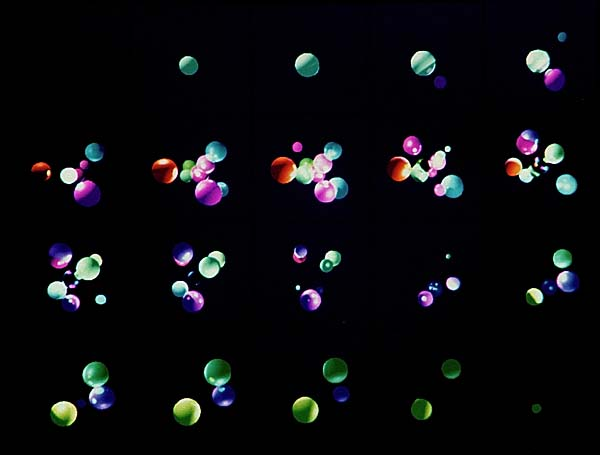Figure 5.4a
Resulting Image Cube Slices
[Sliced 4D Image Cube of Random 4-Sphere Distribution]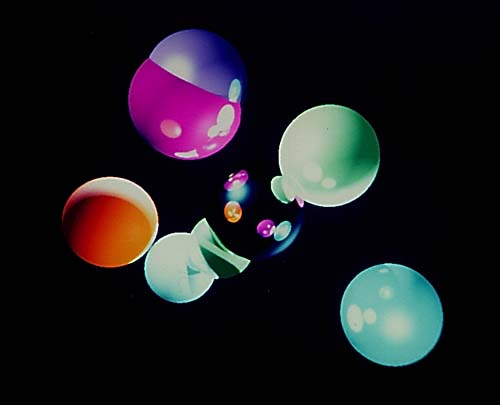Figure 5.4b
Single Slice From Figure 5.4a
[Sliced 4D Image Cube of Random 4-Sphere Distribution]

Figure 5.5 is the sliced image cube of sixteen four-spheres of radius 0.5 positioned at the vertices of a four-cube at locations <±1.25, ±1.25, ±1.25, ±1.25>.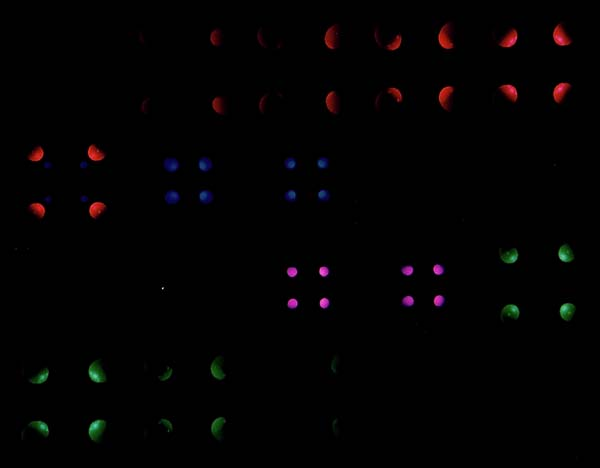Figure 5.5
Sliced Image of 16 4-Spheres Placed at 4-Cube Vertices

Figure 5.6 is similar to figure 5.5, except that four-tetrahedrons are placed at the vertices rather than four-spheres. The four-tetrahedrons are oriented so that the normal of each four-tetrahedron is aimed at the center of the four-cube, and the four-tetrahedron vertices lie on the four-cube edges.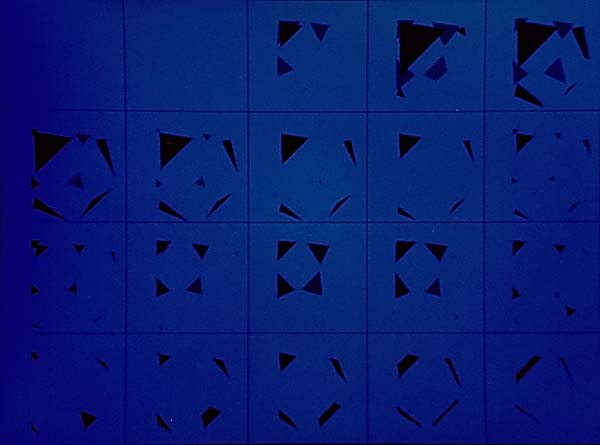Figure 5.6
Sliced Image of 16 4-Tetrahedrons Placed at 4-Cube Vertices

# Chapter 6 Conclusion

The previous chapters explored two approaches to the task of rendering four-dimensional images: wireframe display and raytracing. Both techniques have advantages and disadvantages over the other; e.g. wireframe display is the only real solution to rendering four-space curves. It also allows for rapid display of a four-dimensional structure.

Raytracing, on the other hand, allows the user to view surfaces and solids in and of four dimensions. It also provides other important visual cues, such as shadows, highlights, and reflections. In addition, the output images make it clear which parts are solids projected from four-space; the wireframe approach is subject to ambiguity in the projected image.

## 6.1 Research Conclusions

This research began with the goal of visualizing four-dimensional structures in four dimensions. While several techniques exist (and many more are currently being developed) to visualize four dimensional data as 3D scalar fields, there are few techniques that exist to visualize four-space geometry.

There are, in fact, several 4D wireframe display programs; the earliest documented was written around 1967. The wireframe display program presented in this paper combines the wireframe display with the viewing model presented in [Foley 87], which is a simple and efficient method of projection. In addition, the program written for this research allows for the 4D depth-cueing of the display data, the interactive manipulation of the 4D object, and the interactive selection of the projection modes.

The most promising field of application for this research is the field of Computer-Aided Geometric Design, for the use of displaying curves and surfaces in four dimensions. The wireframe viewer has been used to view 4D spline curves and has displayed artifacts that were not obvious with other methods (see figure 4.15).

The raytracer written for this research implements the four-sphere, the four-tetrahedron, and the four-parallelepiped. It handles point & directional lighting, reflection, refraction, plus ambient, diffuse and specular lighting.

The primary catch with four-dimensional raytracing is the fact that the resulting image is a three-dimensional voxel field, which (for “interesting” images) will have a complex internal structure that is difficult to visualize with current techniques.

## 6.2 Future Research Areas

There's a lot of room for expansion of the 4D raytracer. One obvious area is the inclusion of additional fundamental objects for the raytracer. As mentioned earlier, all 4D objects can be represented with a mesh of tessellating tetrahedra, but this is quite expensive in terms of both storage and time. All that is really needed for a new four-dimensional object is an implicit equation of its hypersurface. The four-dimensional ray equation can be plugged into the implicit object equation to yield the equation for the intersection points. In the case of multiple intersections, the closest intersection point is selected.

In addition, the display of the resulting voxel field could well bear some research. Most visualization techniques work on a 3D space of scalar data; it would be useful if some techniques existed to display a 3D field of RGB data.

The voxel field generated by the raytracer is somewhat different from other fields more often associated with four-dimensional visualization, which are often amorphous fields of scalar values. The output voxel fields of the raytracer are characterized by the following properties:

1. Internal boundaries are well-defined, corresponding to projected objects.
2. There can be quite a lot of different internal solids, often intersecting.
3. Each voxel is assigned an RGB triple.

In order to further understand the 4D images, stereo display techniques for both the wireframe display and the raytrace output may prove useful. There are problems with stereo displays of higher dimensions, primarily the extra degree of parallax, but there may be ways to solve these. See [Brisson 78] for an example of 4D stereograms.

Abbott 52
Edwin A. Abbott, Flatland, Dover Publications, Inc., New York NY, 1952.
Banchoff 90
Thomas F. Banchoff, Beyond the Third Dimension, Scientific American Library, New York NY, 1990.
Barnhill 84
R. E. Barnhill and F. F. Little, “Three– and Four–Dimensional Surfaces,” The Rocky Mountain Journal of Mathematics 14(1), Winter 1984, pp 77-102.
Brisson 78
edited by David W. Brisson, “Visual Comprehension of n-Dimensions,” Hypergraphics: Visualizing Complex Relationships in Art, Science & Technology, Westview Press, Boulder CO, 1978, pp 109-146.
Carey 87
Scott A. Carey, Robert P. Burton, and Douglas M. Campbell, “Shades of a Higher Dimension,” Computer Graphics World, October 1987, pp 93-94.
Chen 85
L. Chen, G. Herman, R. Reynolds, J. Udupa, “Surface Shading in the Cuberille Environment,” IEEE Computer Graphics and Applications 5(12), December 1985, pp 33-43.
Chen 90
Chao-Chi Chen, “Interpolation of Orientation Matrices Using Sphere Splines in Computer Animation,” Master's Thesis, Computer Science Department, Arizona State University, December 1990.
Dewdney 84
A. K. Dewdney, The Planiverse: Computer Contact with a Two-Dimensional World, Poseidon Press, New York NY, 1984.
Dewdney 86
A. K. Dewdney, “Computer Recreations,” Scientific American, April 1986, pp 14-23.
Drebin 88
Robert Drebin, Loren Carpenter, Pat Hanrahan, “Volume Rendering,” Computer Graphics 22(4), August 1988, pp 65-74.
Farin 88
Gerald Farin, Curves and Surfaces for Computer Aided Geometric Design, Academic Press, Inc., San Diego CA, 1988, pp 19,238.
Foley 87
Thomas A. Foley, Gregory M. Nielson, “Practical Techniques for Producing 3D Graphical Images,” VMEbus Systems, November-December 1987, pp 65-73.
Frieder 85
G. Frieder, D. Gordon, R. Reynolds, “Back-to-Front Display of Voxel-Based Objects,” IEEE Computer Graphics and Applications 5(1), January 1985, pp 52-60.
Glassner 90
Andrew Glassner, Graphics Gems, Academic Press, Inc., San Diego CA, 1990, pp 390-393.
Hall 89
Roy Hall, Illumination and Color in Complex Generated Imagery, Springer-Verlag, New York NY, 1989, p 153.
Hill 90
Francis S. Hill, Jr., Computer Graphics, Macmillan Publishing Co., New York NY, 1990.
Kajiya 84
James Kajiya, Brian Von Herzen, “Ray Tracing Volume Densities,” Computer Graphics 18(3), July 1984, pp 165-174.
Lorensen 87
William Lorensen, Harvey Cline, “Marching Cubes: A High Resolution 3D Surface Construction Algorithm,” Computer Graphics 21(4), July 1987, pp 163-169.
Noll 67
Michael A. Noll, “A Computer Technique for Displaying n-Dimensional Hyperobjects,” Communications of the ACM 10(8), August 1967, pp 469-473.
Sabella 88
Paolo Sabella, “A Rendering Algorithm for Visualizing 3D Scalar Fields,” Computer Graphics 22(4), August 1988, pp 51-55.
Steiner 87
K. Victor Steiner and Robert P. Burton, “Hidden Volumes: The 4th Dimension,” Computer Graphics World, February 1987, pp 71-74.
Whitted 80
Turner Whitted, “An Improved Illumination Model for Shaded Display,” Communications of the ACM 23(6), June 1980, pp 343-349.

# Appendix AImplementation Notes

The programs written for this research are:

 wire4 4D Wireframe Display Program ray4 4D Raytracer r4toiff Ray4 to Interchange File Format (IFF) Image r4tosgi Ray4 to SGI Display

The current version of these programs is available on GitHub at http://github.com/hollasch/ray4/.

## The wire4 Program

The wire4 program runs on the Silicon Graphics Iris 3130 workstation and uses the Silicon Graphics GL display language. The input file specifies the following data:

• 3D Viewing Parameters: From, To, Up, View-Angle
• 4D Viewing Parameters: From, To, Up, Over, View-Angle
• Vertex List
• Edge List
• Edge Color Palette
• Depthcue Parameters: Minimum & Maximum Distance
• Depthcue Parameters: Near & Far Colors, Depthcue Levels

wire4 reads the input file and displays the wireframe with the initial viewing parameters. Since only the viewpoints are rotated, the 4D and 3D distances from the from-point to the to-point are constant. The user has interactive control over the following:

• Rotation in 3D (3 Planes)
• Rotation in 4D (6 Planes)
• 4D Projection Type (Parallel or Perspective)
• 3D Projection Type (Parallel or Perspective)
• Depthcue On/Off
• 3D Projection Cube Display On/Off

## The ray4 Program

ray4 runs on both the Commodore Amiga and most Unix platforms. Since the output is sent to a file, this program is device independent.

The input file contains the following information:

• Global Ambient Light
• Background Color
• Maximum Raytrace Depth
• 4D Viewing Parameters:
• From Point,
• To Point,
• Up Vector,
• Over Vector,
• Viewing-Angle
• Light Sources:
• Point,
• Directional
• Attribute Descriptions
• Ambient Color,
• Diffuse Color,
• Specular Color,
• Transparent Color,
• Phong Specular Exponent,
• Index of Refraction,
• Reflection
• Object Definitions
• Hyperspheres,
• Tetrahedrons,
• Parallelepipeds

In addition to the scene description, ray4 takes the following command-line arguments which govern the resolution of the output image:

• Aspect Ratios (X:Y:Z)
• Image Resolution (X:Y:Z)
• Scan Range (Xmin-Xmax:Ymin-Ymax:Zmin-Zmax)
• Scene Description Filename
• Output Image Filename